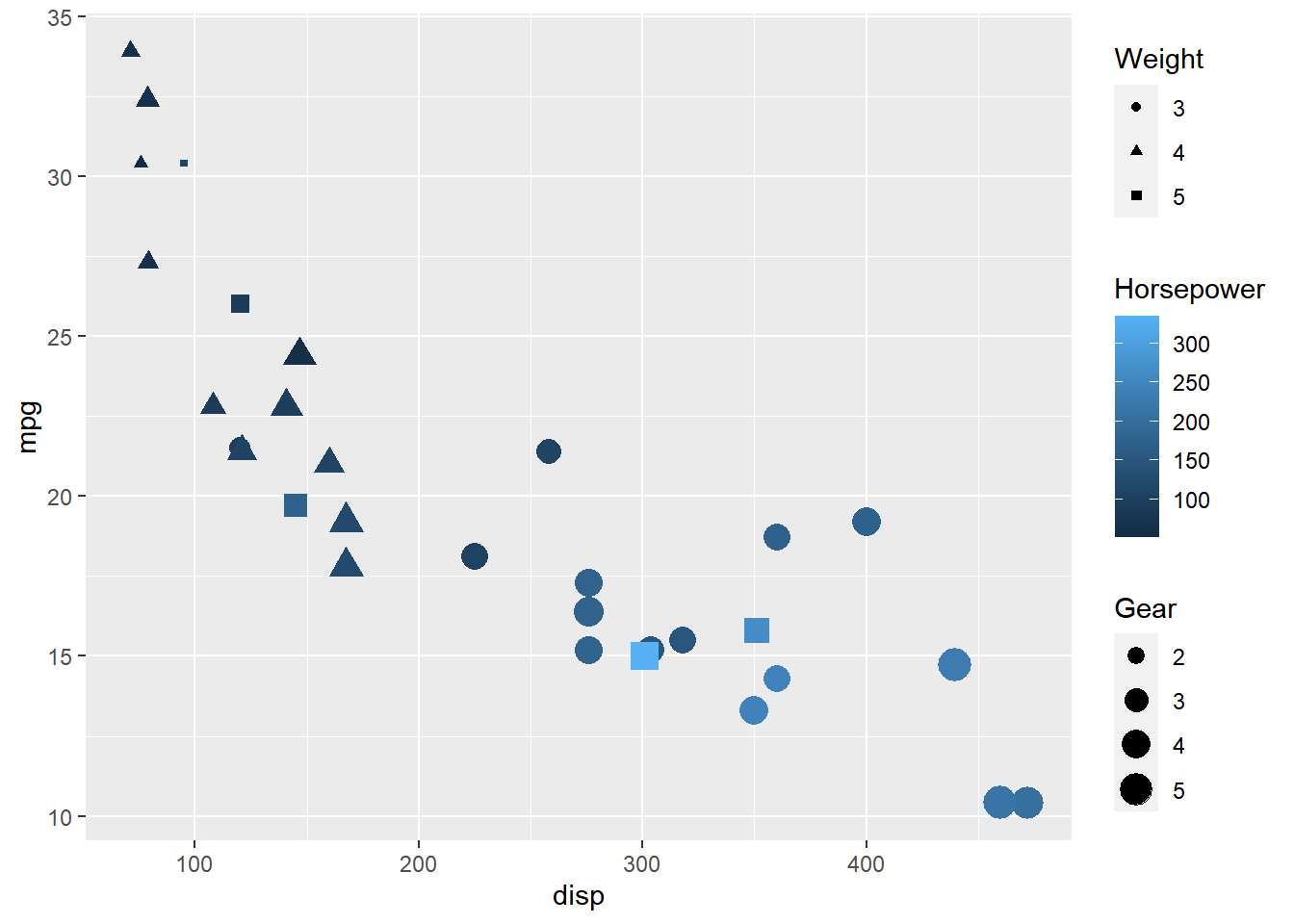# Chapter 12 Modify Legend

In this chapter, we will focus on modifying the appearance of legend of plots when the aesthetics are mapped to variables.

## 12.1 Color

We will learn to modify the following when `color` is mapped to categorical variables:

• title
• breaks
• limits
• labels
• values

### Basic Plot

Let us start with a scatter plot examining the relationship between displacement and miles per gallon from the mtcars data set. We will map the color of the points to the `cyl` variable.

``````ggplot(mtcars) +
geom_point(aes(disp, mpg, color = factor(cyl)))``````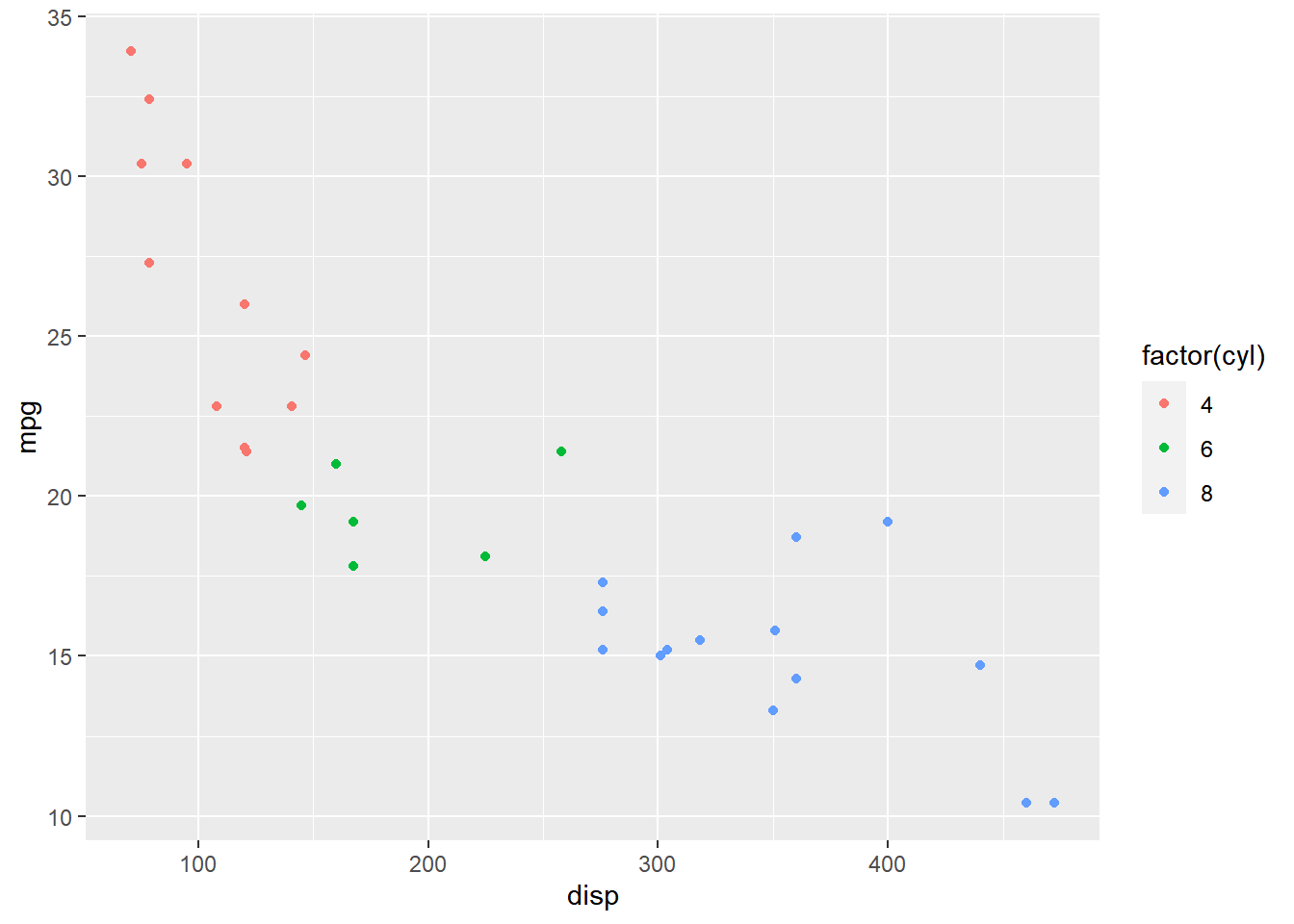As you can see, the legend acts as a guide for the `color` aesthetic. Now, let us learn to modify the different aspects of the legend.

### Values

To change the default colors in the legend, use the `values` argument and supply a character vector of color names. The number of colors specified must be equal to the number of levels in the categorical variable mapped. In the below example, `cyl` has 3 levels (4, 6, 8) and hence we have specified 3 colors.

``````ggplot(mtcars) +
geom_point(aes(disp, mpg, color = factor(cyl))) +
scale_color_manual(values = c("red", "blue", "green"))``````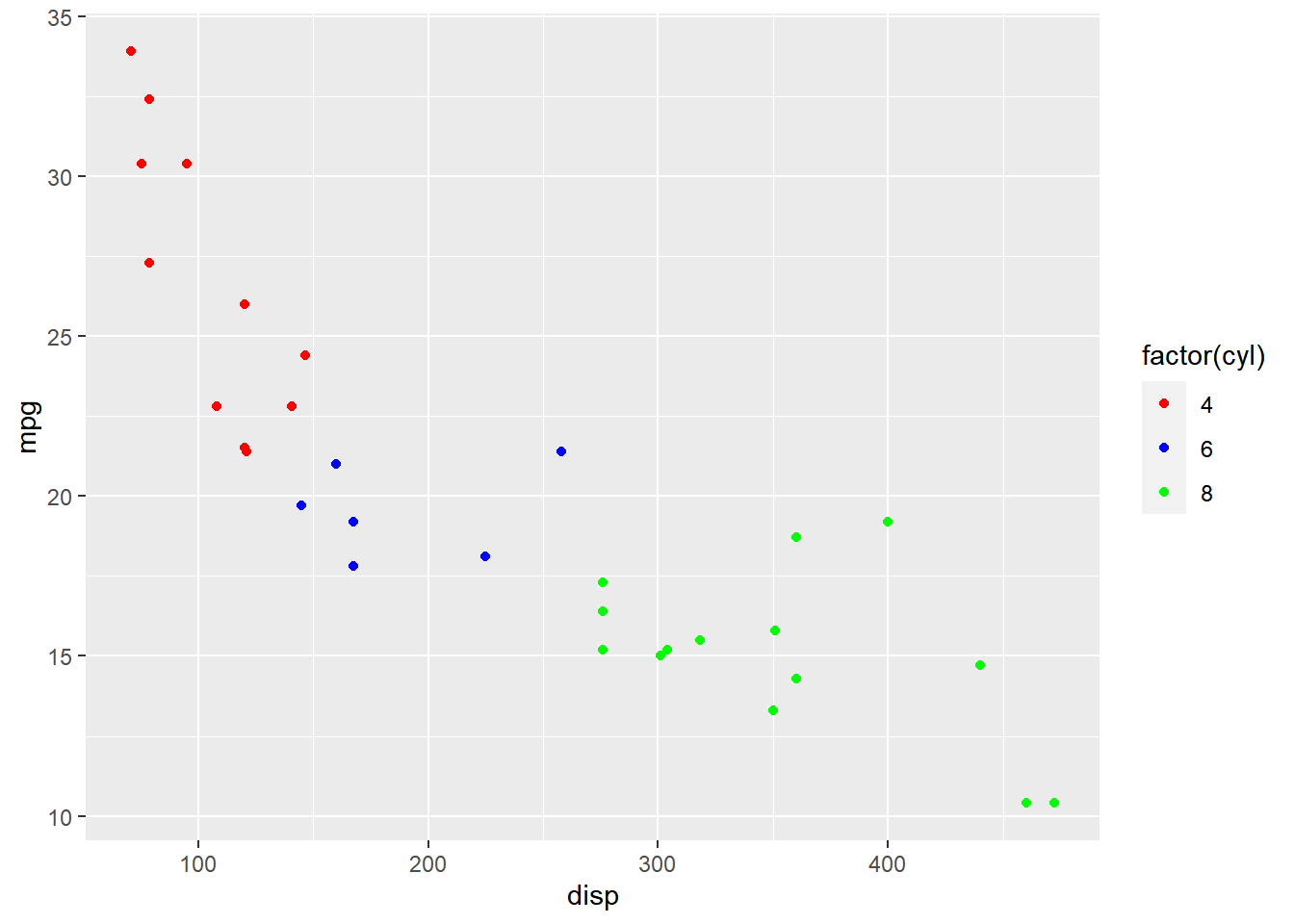### Title

In the previous example, the title of the legend (`factor(cyl)`) is not very intuitive. If the user does not know the underlying data, they will not be able to make any sense out of it. Let us change it to `Cylinders` using the `name` argument.

``````ggplot(mtcars) +
geom_point(aes(disp, mpg, color = factor(cyl))) +
scale_color_manual(name = "Cylinders",
values = c("red", "blue", "green"))``````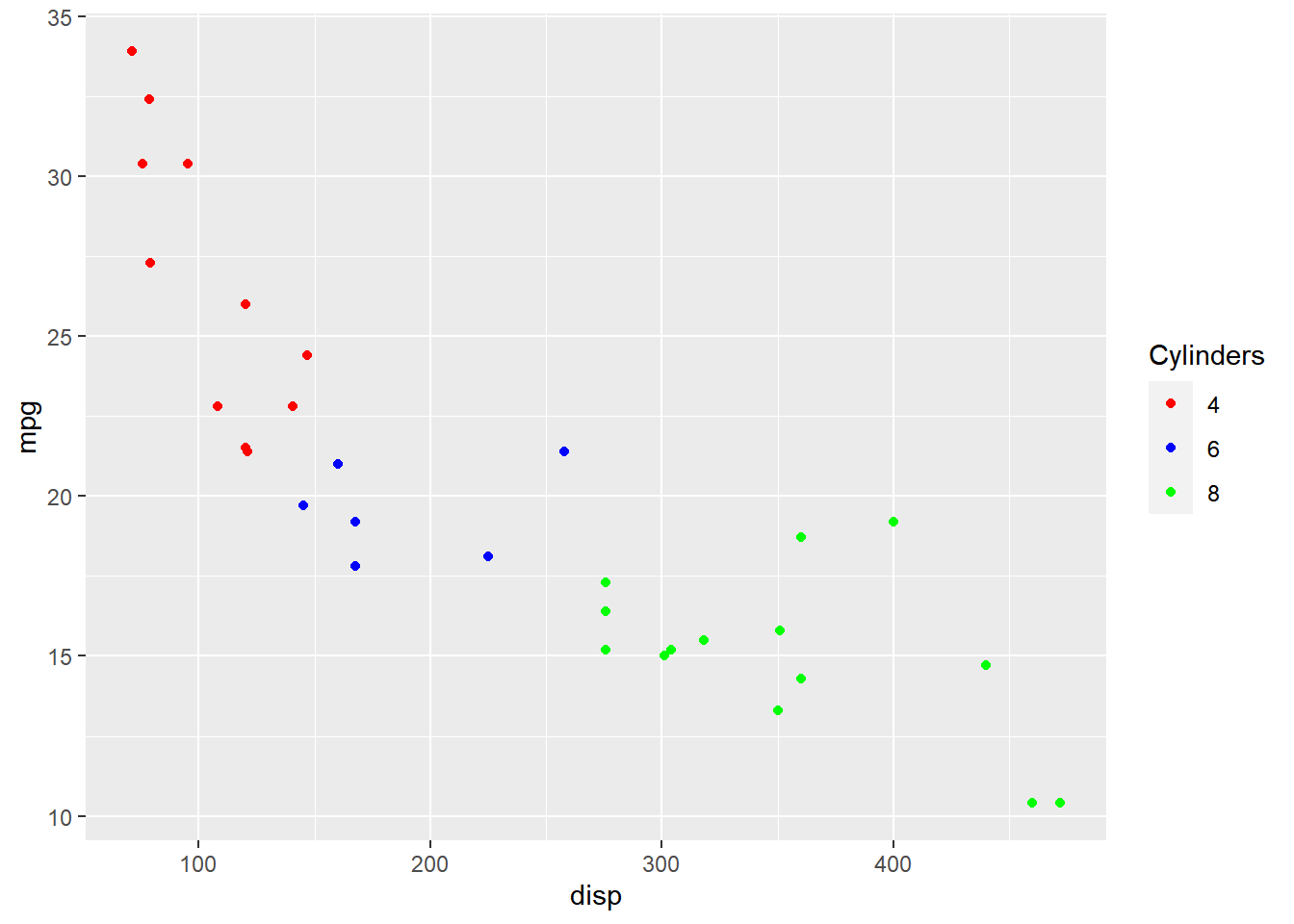Now, the user will know that the different colors represent number of cylinders in the car.

### Limits

Let us assume that we want to modify the data to be displayed i.e. instead of examining the relationship between mileage and displacement for all cars, we desire to look at only cars with at least 6 cylinders. One way to approach this would be to filter the data using `filter` from dplyr and then visualize it. Instead, we will use the `limits` argument and filter the data for visualization.

``````ggplot(mtcars) +
geom_point(aes(disp, mpg, color = factor(cyl))) +
scale_color_manual(values = c("red", "blue", "green"), limits = c(6, 8))``````
``````## Warning: Continuous limits supplied to discrete scale.
## Did you mean `limits = factor(...)` or `scale_*_continuous()`?``````
``## Warning: Removed 11 rows containing missing values (geom_point).``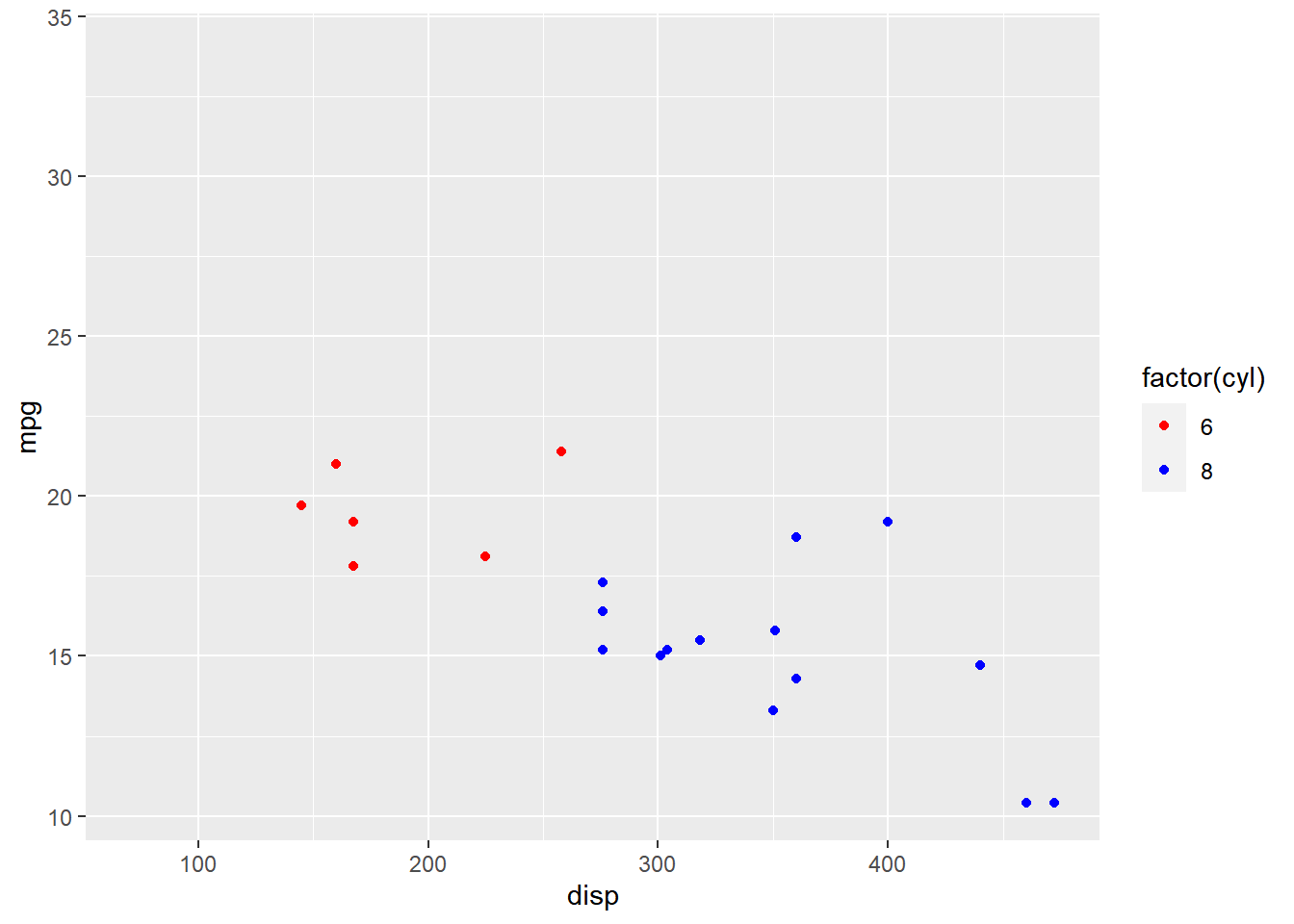As you can see above, `ggplot2` returns a warning message indicating data related to 4 cylinders has been dropped. If you observe the legend, it now represents only 4 and 6 cylinders.

### Labels

The labels in the legend can be modified using the `labels` argument. Let us change the labels to `Four`, `Six` and `Eight` in the next example. Ensure that the labels are intuitive and easy to interpret for the end user of the plot.

``````ggplot(mtcars) +
geom_point(aes(disp, mpg, color = factor(cyl))) +
scale_color_manual(values = c("red", "blue", "green"),
labels = c('Four', 'Six', 'Eight'))``````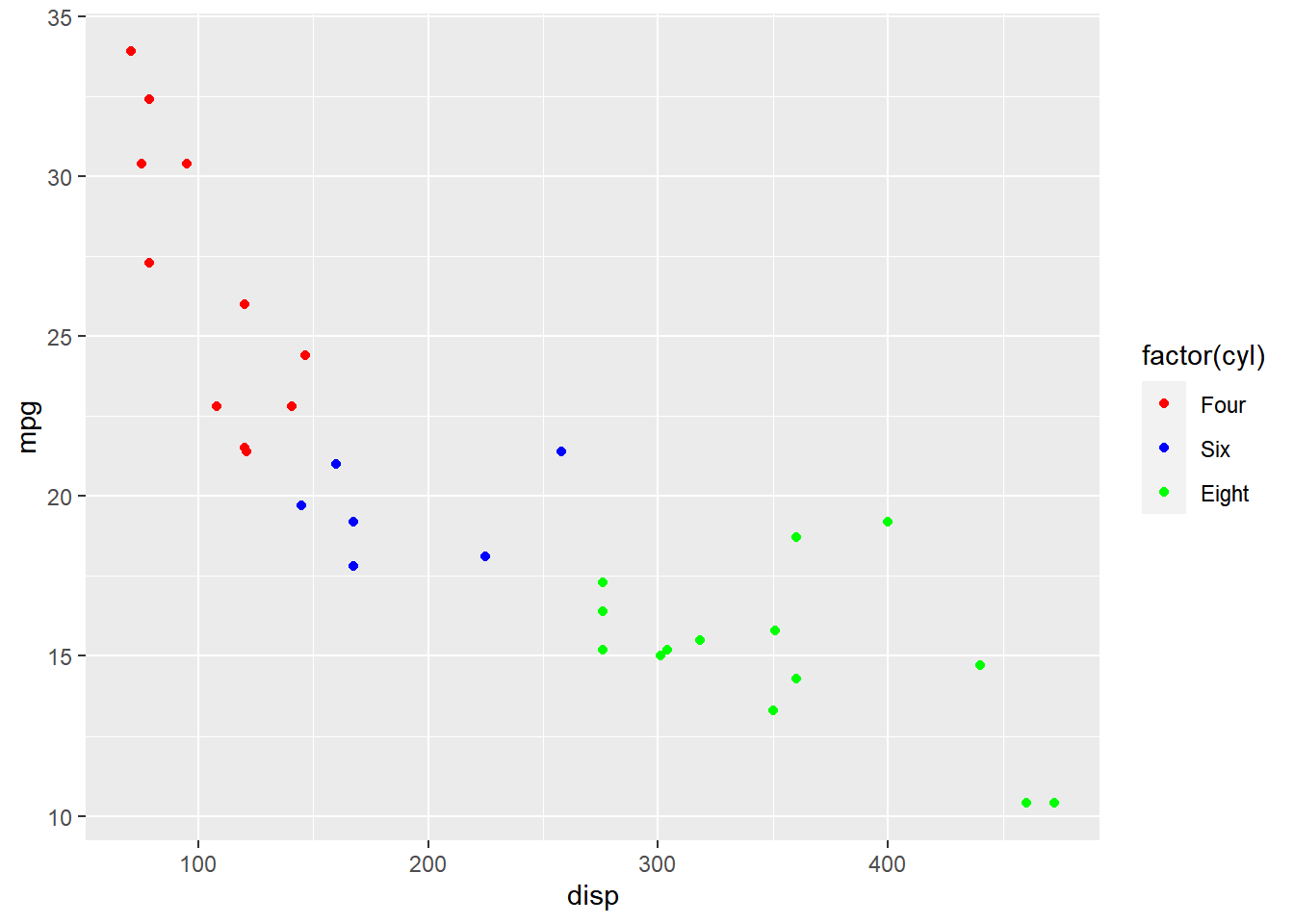### Breaks

When there are large number of levels in the mapped variable, you may not want the labels in the legend to represent all of them. In such cases, we can use the breaks argument and specify the labels to be used. In the below case, we use the `breaks` argument to ensure that the labels in legend represent two levels (4, 8) of the mapped variable.

``````ggplot(mtcars) +
geom_point(aes(disp, mpg, color = factor(cyl))) +
scale_color_manual(values = c("red", "blue", "green"),
breaks = c(4, 8))``````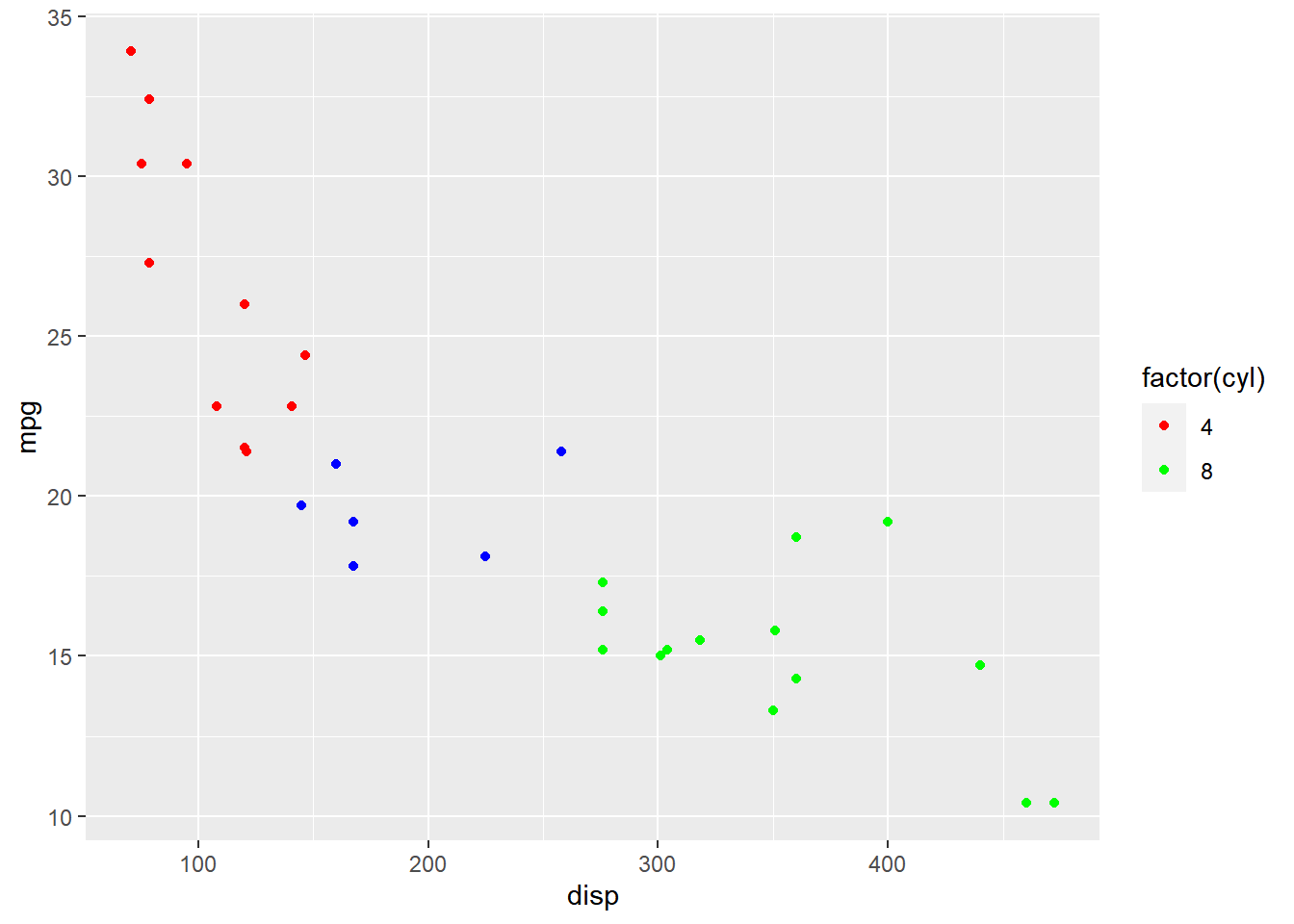### Putting it all together…

``````ggplot(mtcars) +
geom_point(aes(disp, mpg, color = factor(cyl))) +
scale_color_manual(name = "Cylinders", values = c("red", "blue", "green"),
labels = c('Four', 'Six', 'Eight'), limits = c(4, 6, 8), breaks = c(4, 6, 8))``````
``````## Warning: Continuous limits supplied to discrete scale.
## Did you mean `limits = factor(...)` or `scale_*_continuous()`?``````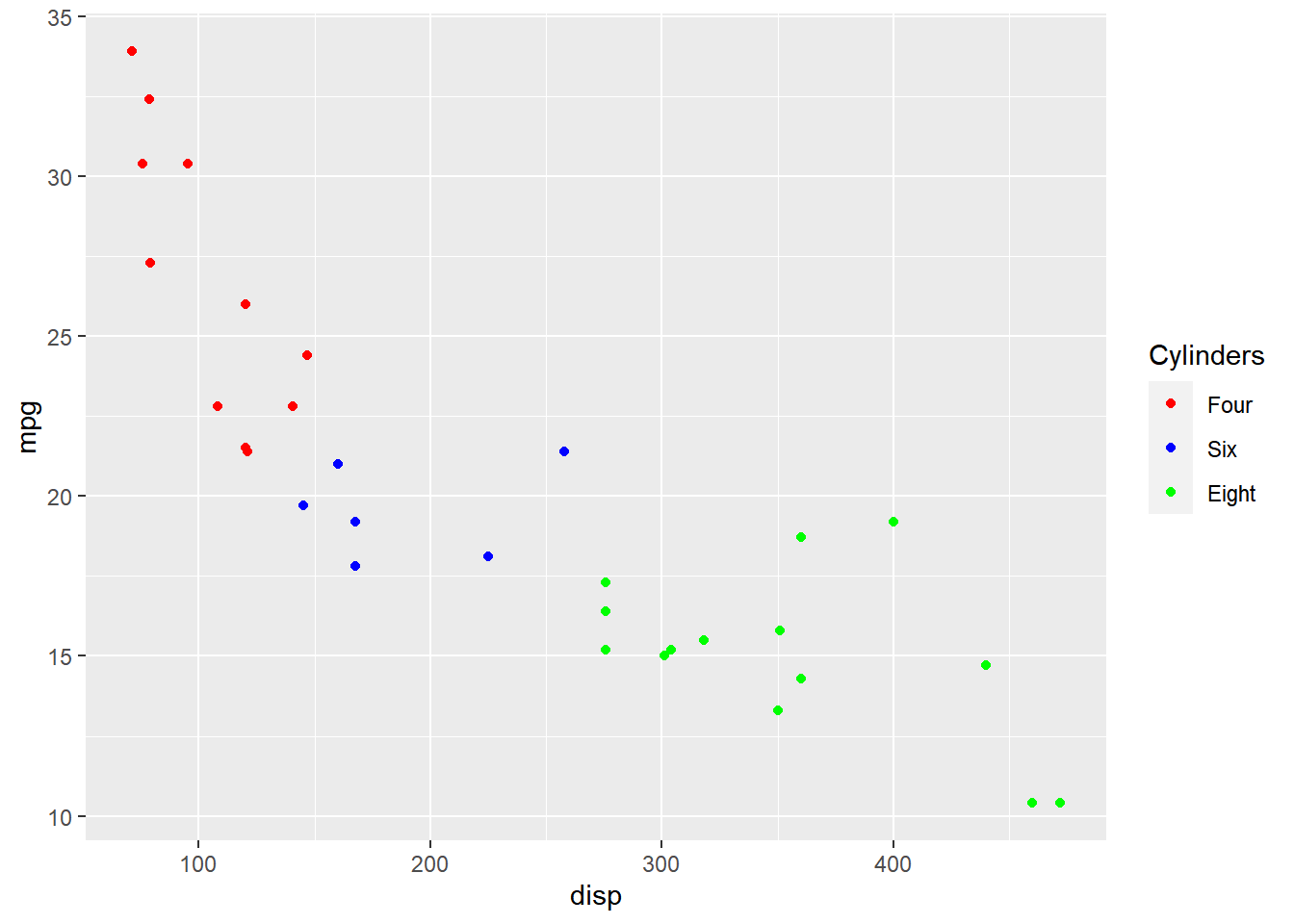## 12.2 Fill

we will learn to modify the following using `scale_fill_manual()` when `fill` is mapped to categorical variables:

• title
• breaks
• limits
• labels
• values

### Plot

Let us start with a scatter plot examining the relationship between displacement and miles per gallon from the mtcars data set. We will map `fill` to the `cyl` variable.

``````ggplot(mtcars) +
geom_point(aes(disp, mpg, fill = factor(cyl)), shape = 22)``````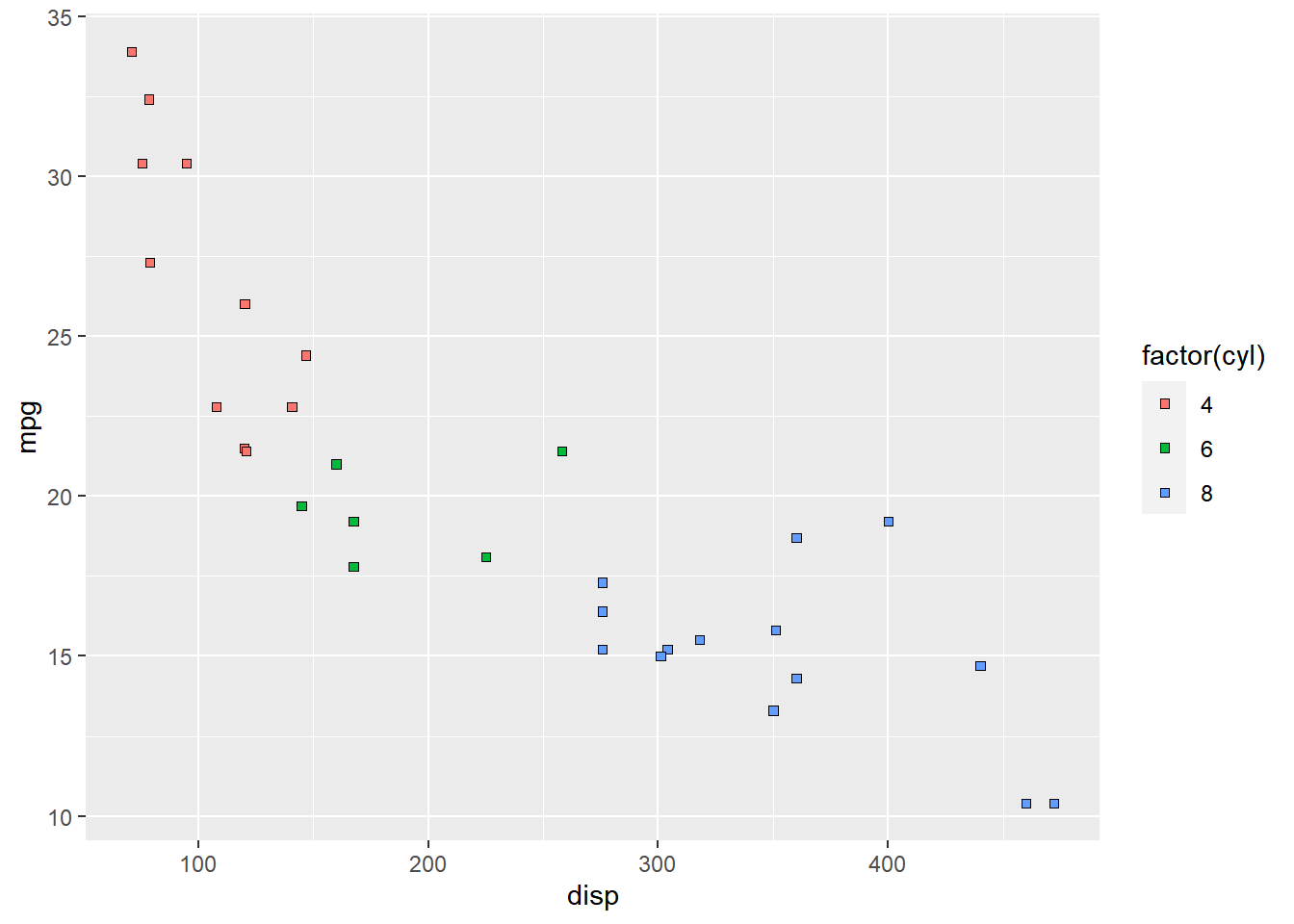As you can see, the legend acts as a guide for the `color` aesthetic. Now, let us learn to modify the different aspects of the legend.

### Title

The title of the legend (`factor(cyl)`) is not very intuitive. If the user does not know the underlying data, they will not be able to make any sense out of it. Let us change it to `Cylinders` using the `name` argument.

``````ggplot(mtcars) +
geom_point(aes(disp, mpg, fill = factor(cyl)), shape = 22) +
scale_fill_manual(name = "Cylinders",
values = c("red", "blue", "green"))``````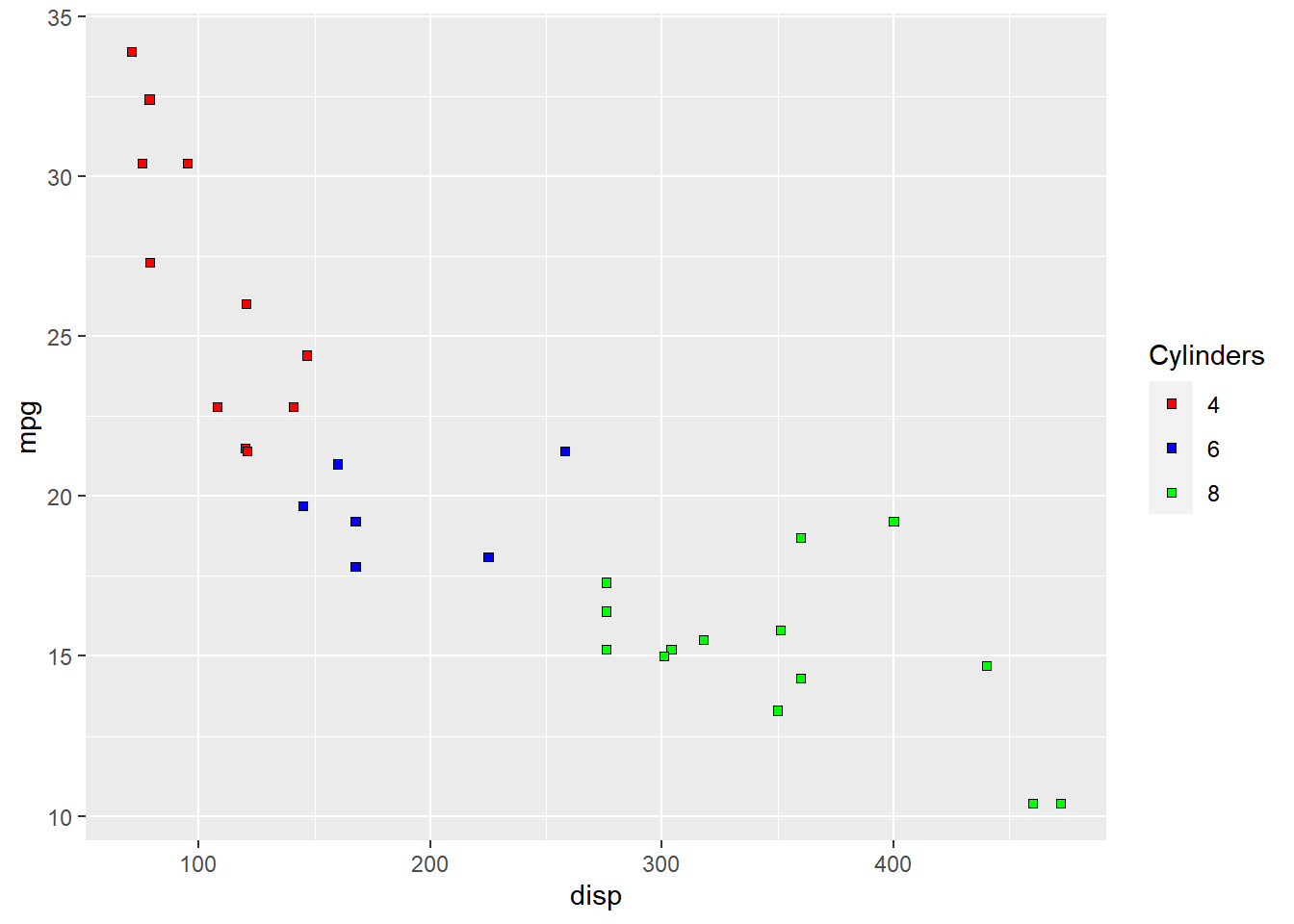### Values

To change the default colors in the legend, use the `values` argument and supply a character vector of color names. The number of colors specified must be equal to the number of levels in the categorical variable mapped. In the below example, `cyl` has 3 levels (4, 6, 8) and hence we have specified 3 colors.

``````ggplot(mtcars) +
geom_point(aes(disp, mpg, fill = factor(cyl)), shape = 22) +
scale_fill_manual(values = c("red", "blue", "green"))``````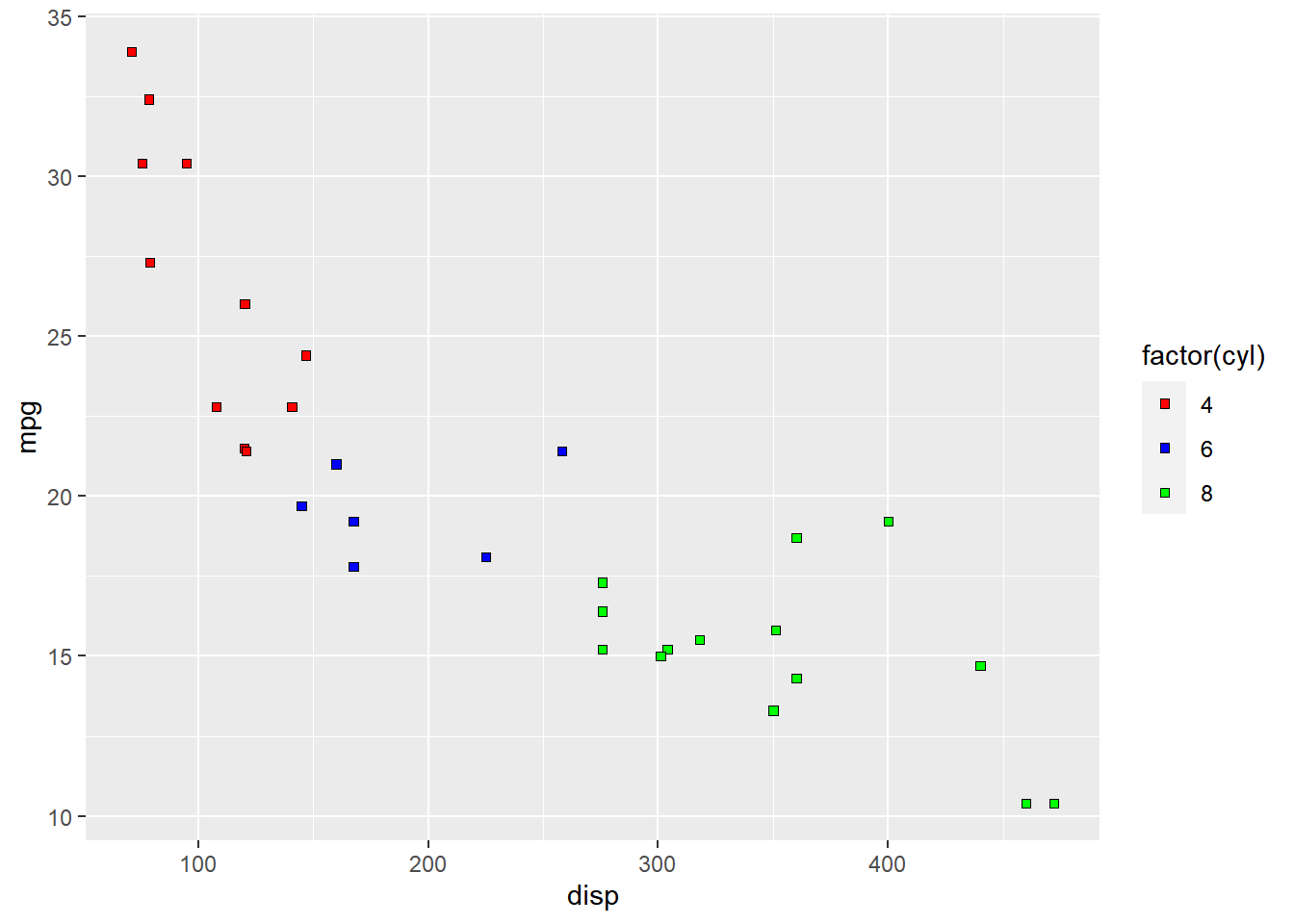### Labels

The labels in the legend can be modified using the `labels` argument. Let us change the labels to `Four`, `Six` and `Eight` in the next example. Ensure that the labels are intuitive and easy to interpret for the end user of the plot.

``````ggplot(mtcars) +
geom_point(aes(disp, mpg, fill = factor(cyl)), shape = 22) +
scale_fill_manual(values = c("red", "blue", "green"),
labels = c('Four', 'Six', 'Eight'))``````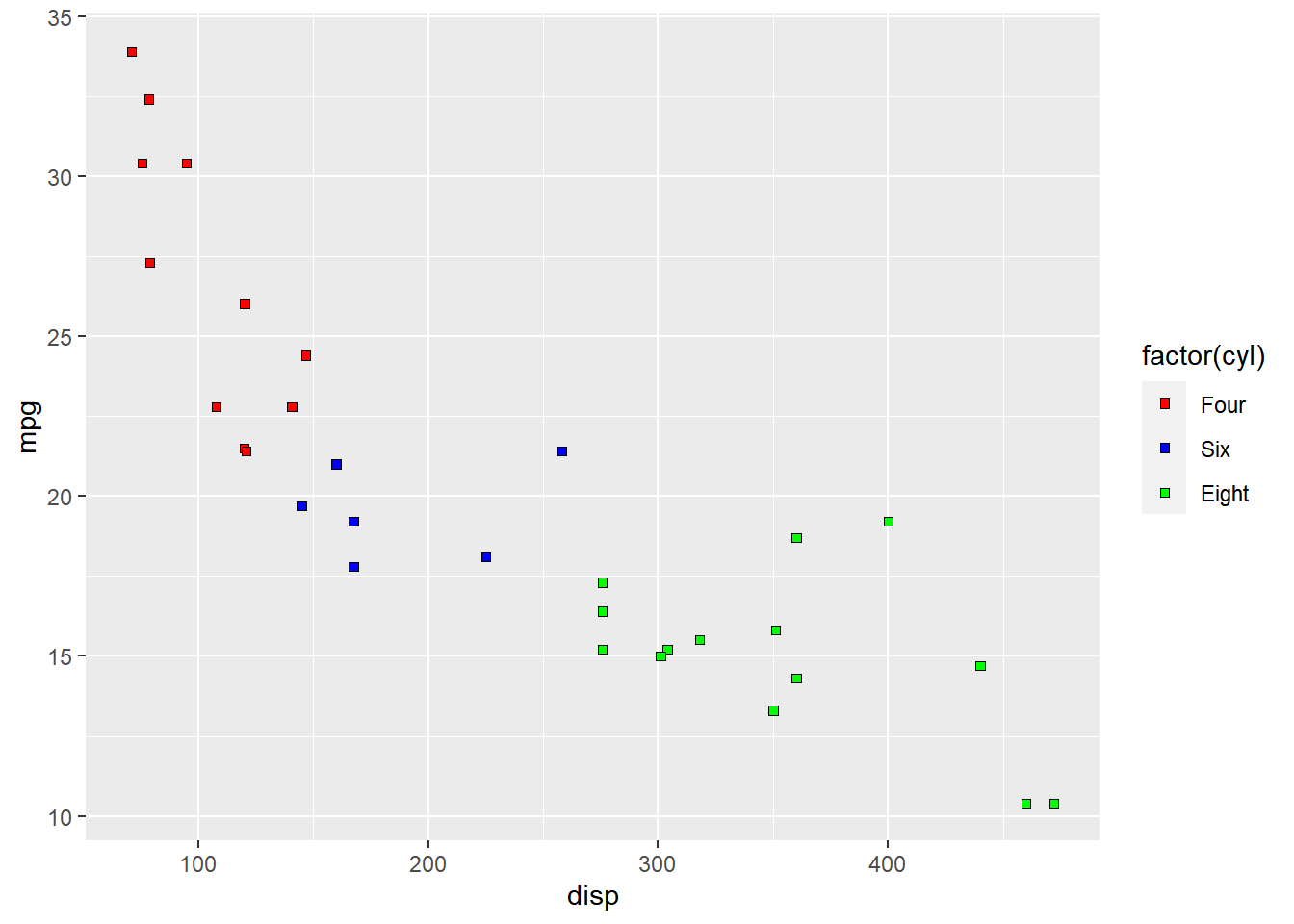### Limits

Let us assume that we want to modify the data to be displayed i.e. instead of examining the relationship between mileage and displacement for all cars, we desire to look at only cars with at least 6 cylinders. One way to approach this would be to filter the data using `filter` from dplyr and then visualize it. Instead, we will use the `limits` argument and filter the data for visualization.

``````ggplot(mtcars) +
geom_point(aes(disp, mpg, fill = factor(cyl)), shape = 22) +
scale_fill_manual(values = c("red", "blue", "green"),
limits = c(6, 8))``````
``````## Warning: Continuous limits supplied to discrete scale.
## Did you mean `limits = factor(...)` or `scale_*_continuous()`?``````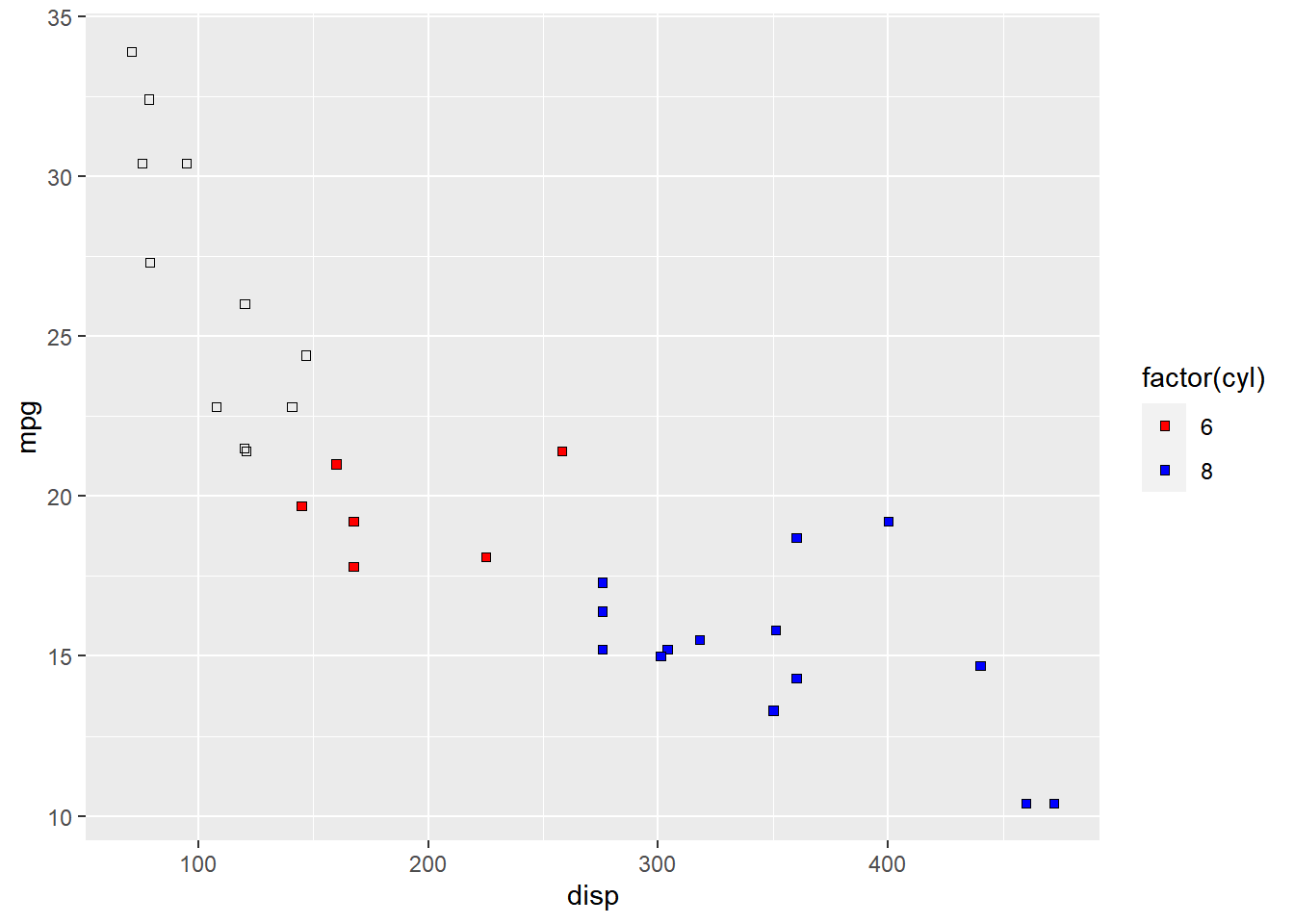As you can see above, `ggplot2` returns a warning message indicating data related to 4 cylinders has been dropped. If you observe the legend, it now represents only 4 and 6 cylinders.

### Breaks

When there are large number of levels in the mapped variable, you may not want the labels in the legend to represent all of them. In such cases, we can use the breaks argument and specify the labels to be used. In the below case, we use the `breaks` argument to ensure that the labels in legend represent two levels (4, 8) of the mapped variable.

``````ggplot(mtcars) +
geom_point(aes(disp, mpg, fill = factor(cyl)), shape = 22) +
scale_fill_manual(values = c("red", "blue", "green"),
breaks = c(4, 8))``````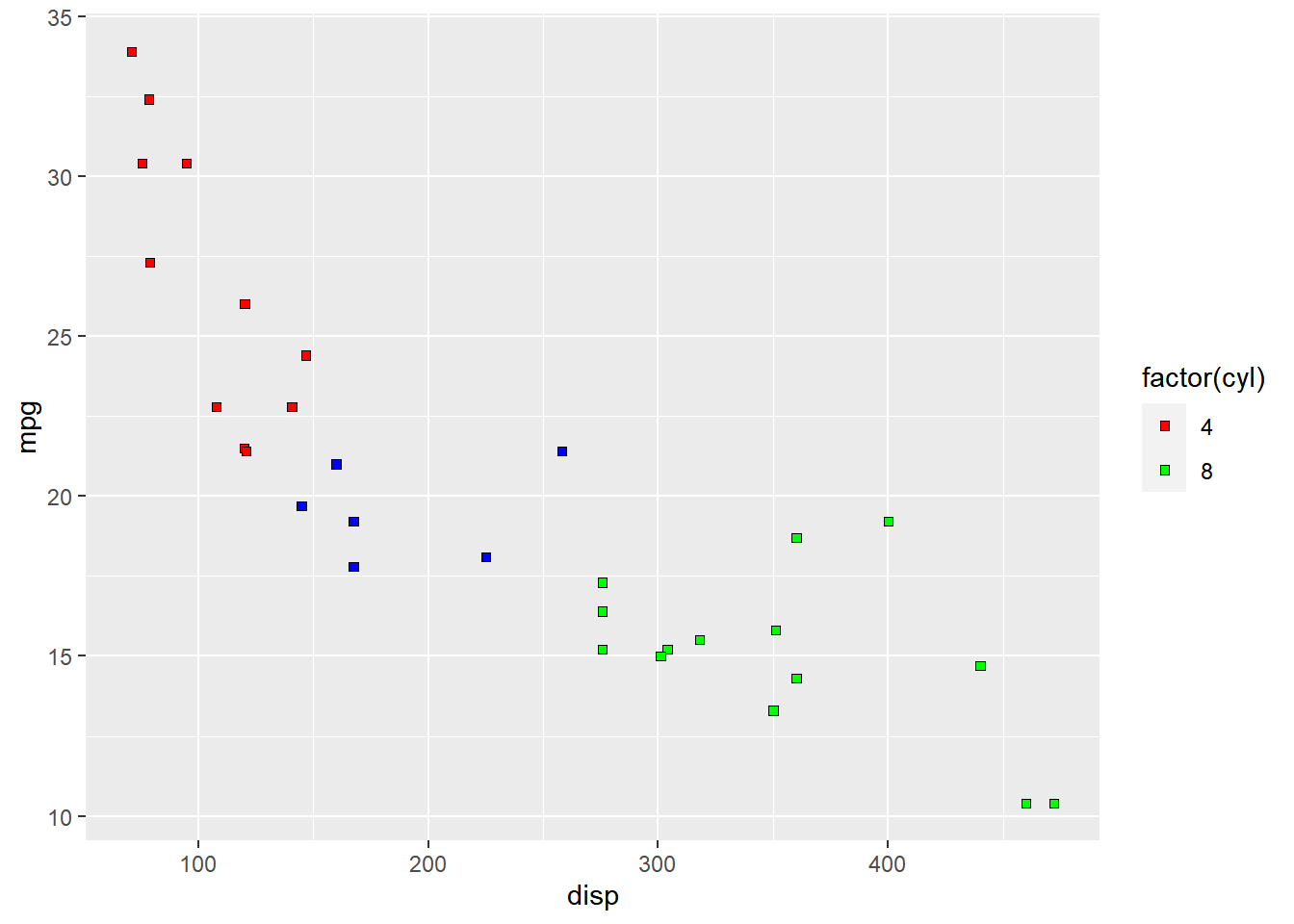### Putting it all together…

``````ggplot(mtcars) +
geom_point(aes(disp, mpg, fill = factor(cyl)), shape = 22) +
scale_fill_manual(name = "Cylinders", values = c("red", "blue", "green"),
labels = c('Four', 'Six', 'Eight'), limits = c(4, 6, 8), breaks = c(4, 6, 8))``````
``````## Warning: Continuous limits supplied to discrete scale.
## Did you mean `limits = factor(...)` or `scale_*_continuous()`?``````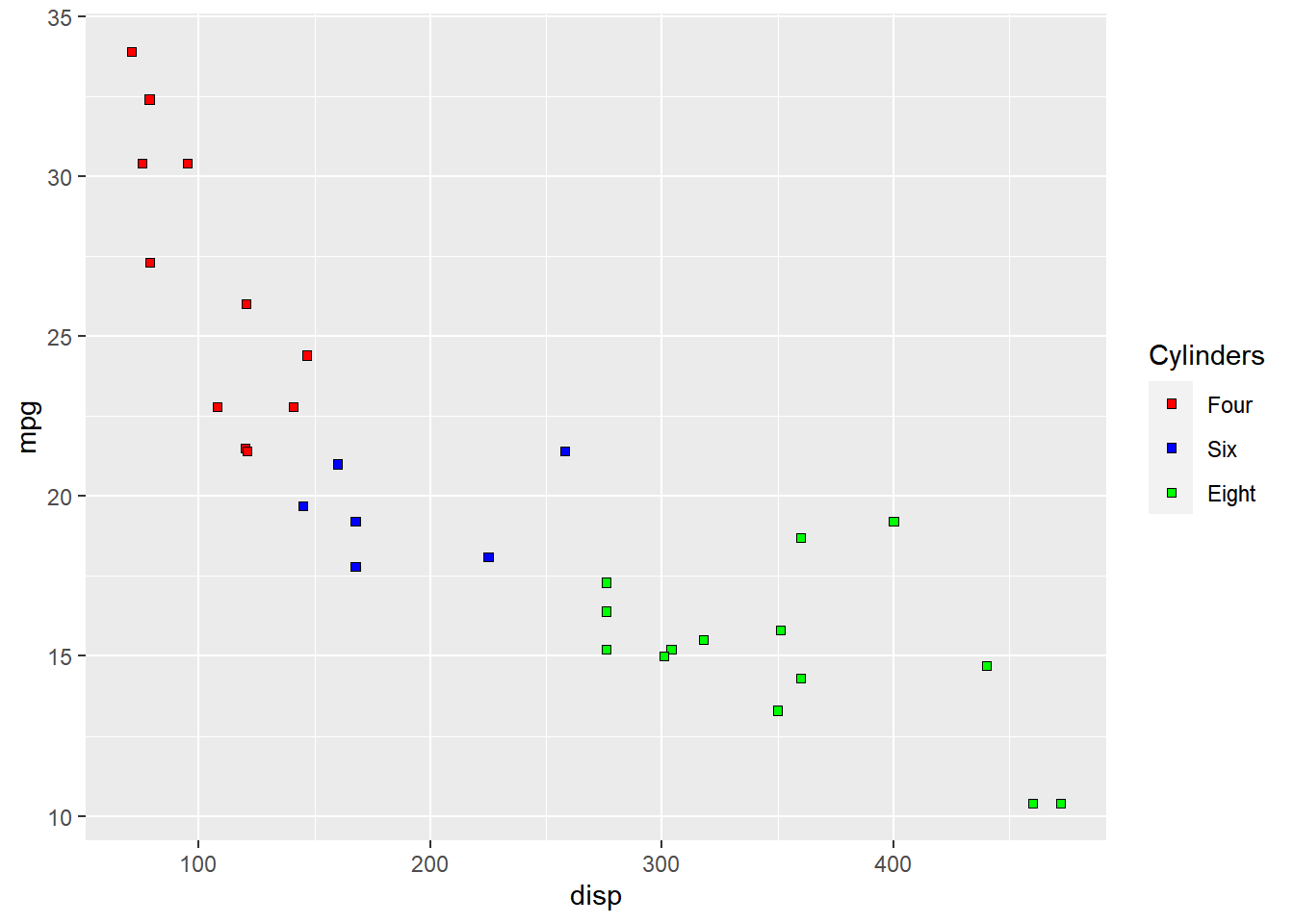## 12.3 Shape

We will learn to modify the following using `scale_shape_manual` when `shape` is mapped to categorical variables:

• title
• breaks
• limits
• labels
• values

### Plot

Let us start with a scatter plot examining the relationship between displacement and miles per gallon from the mtcars data set. We will map the shape of the points to the `cyl` variable.

``````ggplot(mtcars) +
geom_point(aes(disp, mpg, shape = factor(cyl)))``````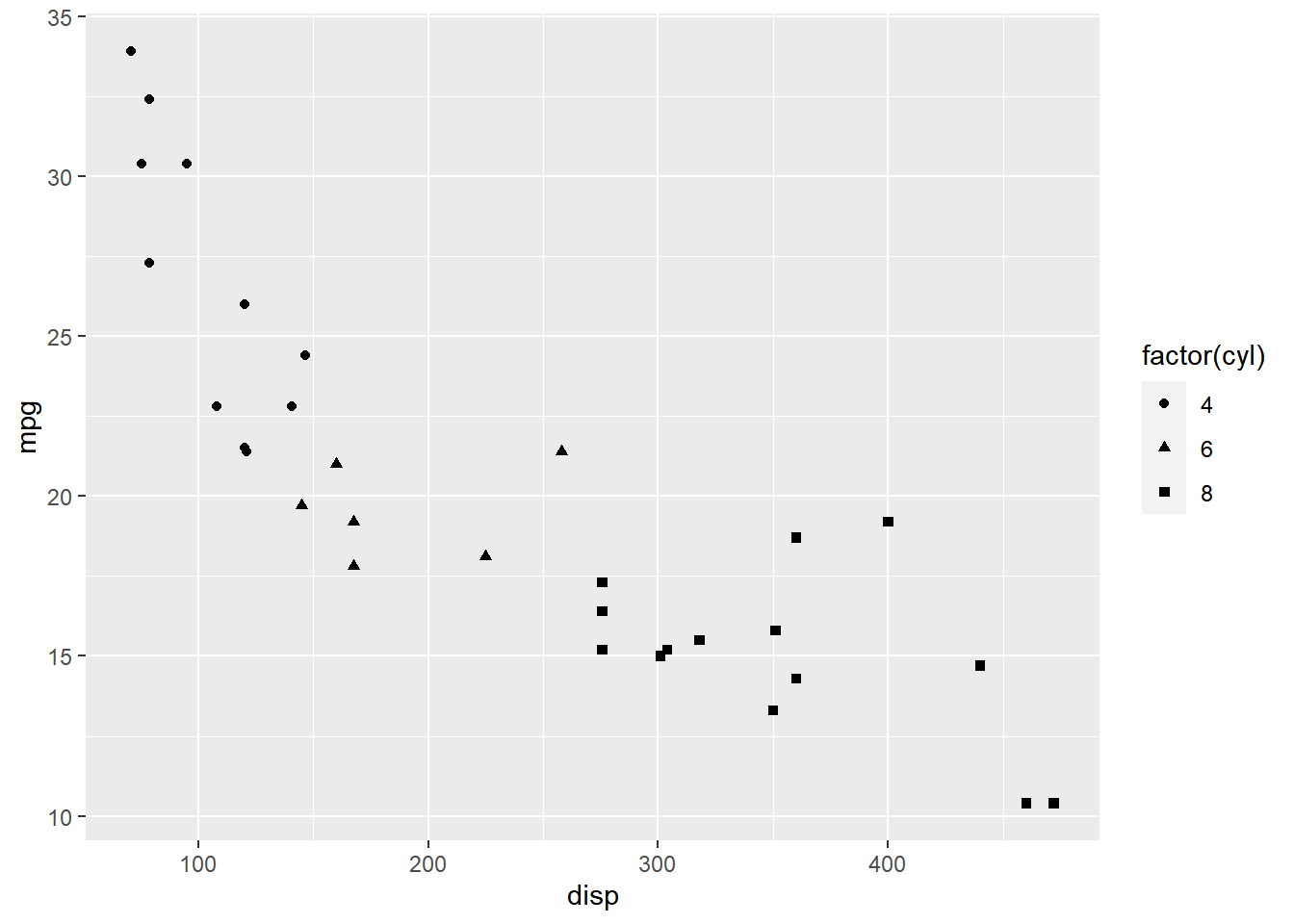As you can see, the legend acts as a guide for the `shape` aesthetic. Now, let us learn to modify the different aspects of the legend.

### Title

The title of the legend (`factor(cyl)`) is not very intuitive. If the user does not know the underlying data, they will not be able to make any sense out of it. Let us change it to `Cylinders` using the `name` argument.

``````ggplot(mtcars) +
geom_point(aes(disp, mpg, shape = factor(cyl))) +
scale_shape_manual(name = "Cylinders", values = c(4, 12, 24))``````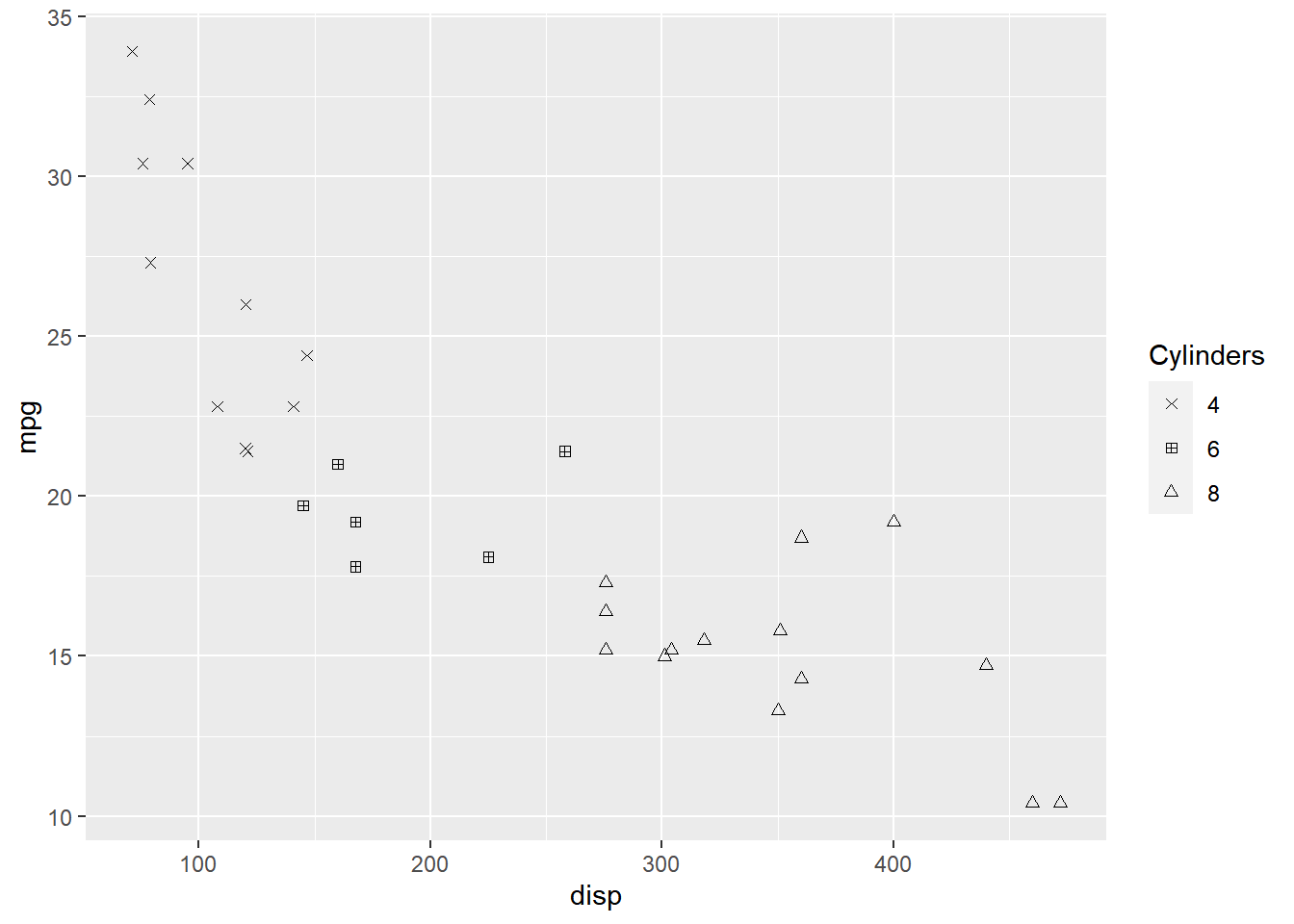If you have mapped shape/size to a discrete variable which has less than six categories, you can use `scale_shape()`.

``````ggplot(mtcars) +
geom_point(aes(disp, mpg, shape = factor(cyl))) +
scale_shape(name = 'Cylinders')``````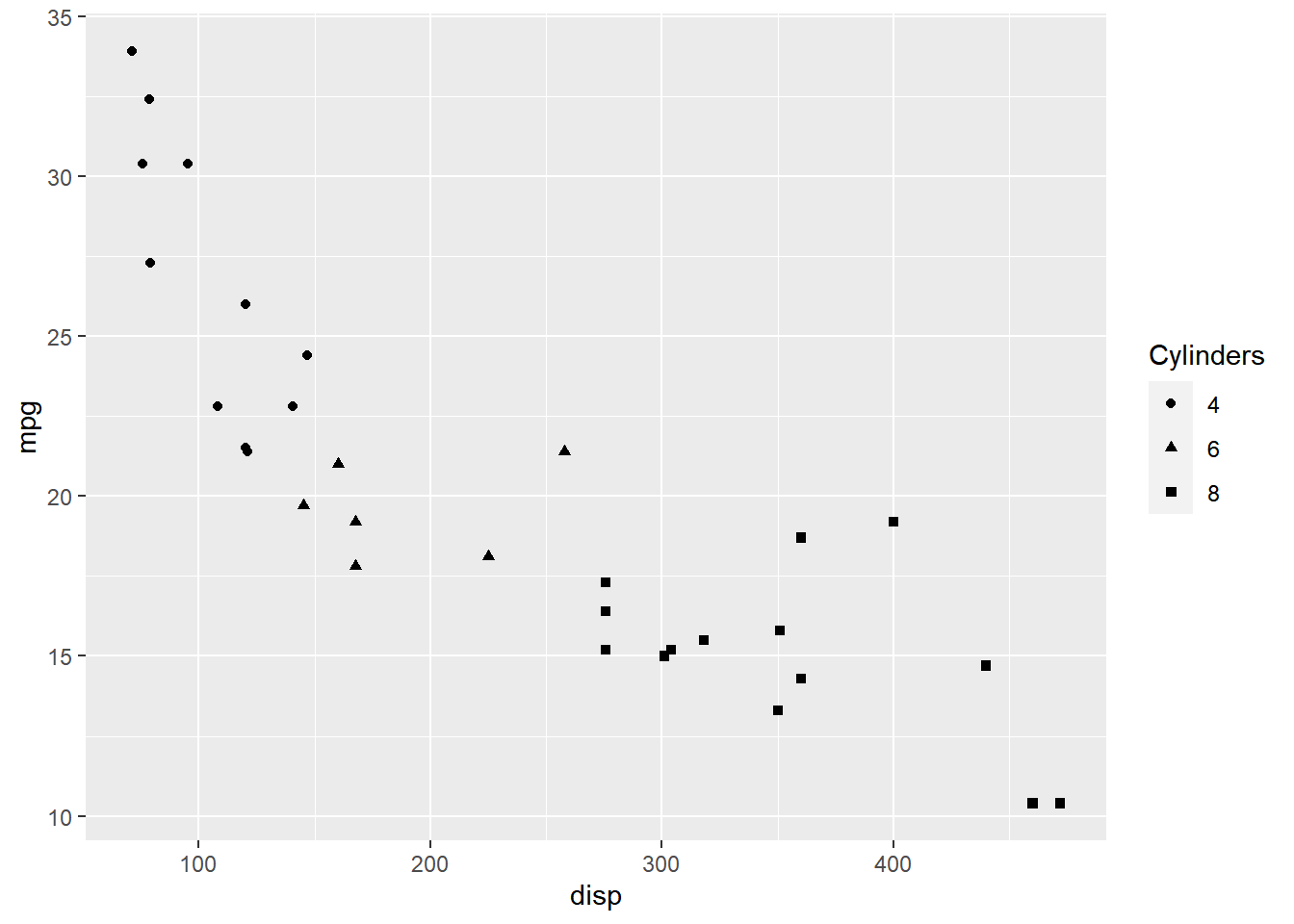### Values

To change the default shapes in the legend, use the `values` argument and supply a numeric vector of shapes. The number of shapes specified must be equal to the number of levels in the categorical variable mapped. In the below example, `cyl` has 3 levels (4, 6, 8) and hence we have specified 3 different shapes.

``````ggplot(mtcars) +
geom_point(aes(disp, mpg, shape = factor(cyl))) +
scale_shape_manual(values = c(4, 12, 24))``````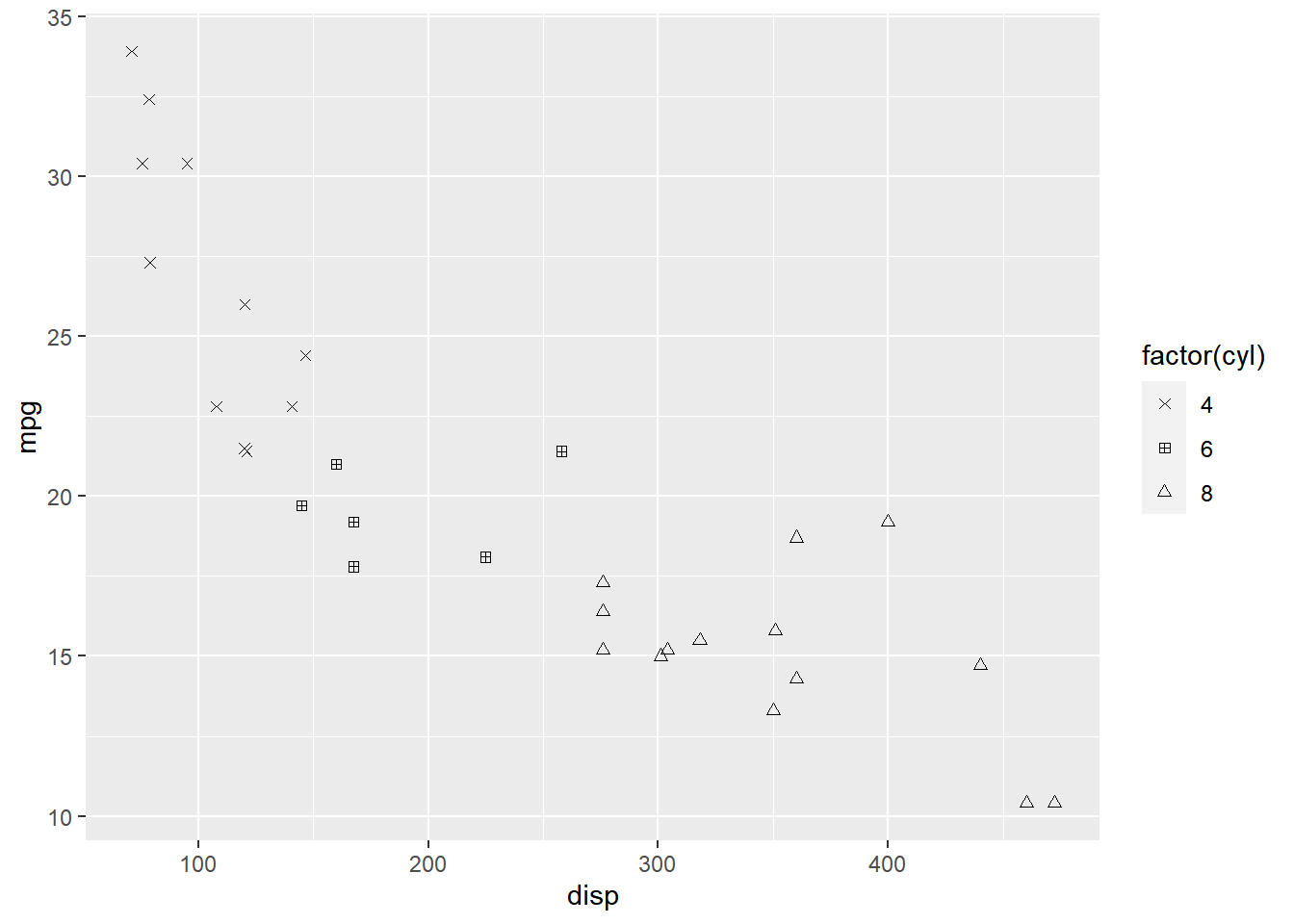### Labels

The labels in the legend can be modified using the `labels` argument. Let us change the labels to `Four`, `Six` and `Eight` in the next example. Ensure that the labels are intuitive and easy to interpret for the end user of the plot.

``````ggplot(mtcars) +
geom_point(aes(disp, mpg, shape = factor(cyl))) +
scale_shape_manual(values = c(4, 12, 24), labels = c('Four', 'Six', 'Eight'))``````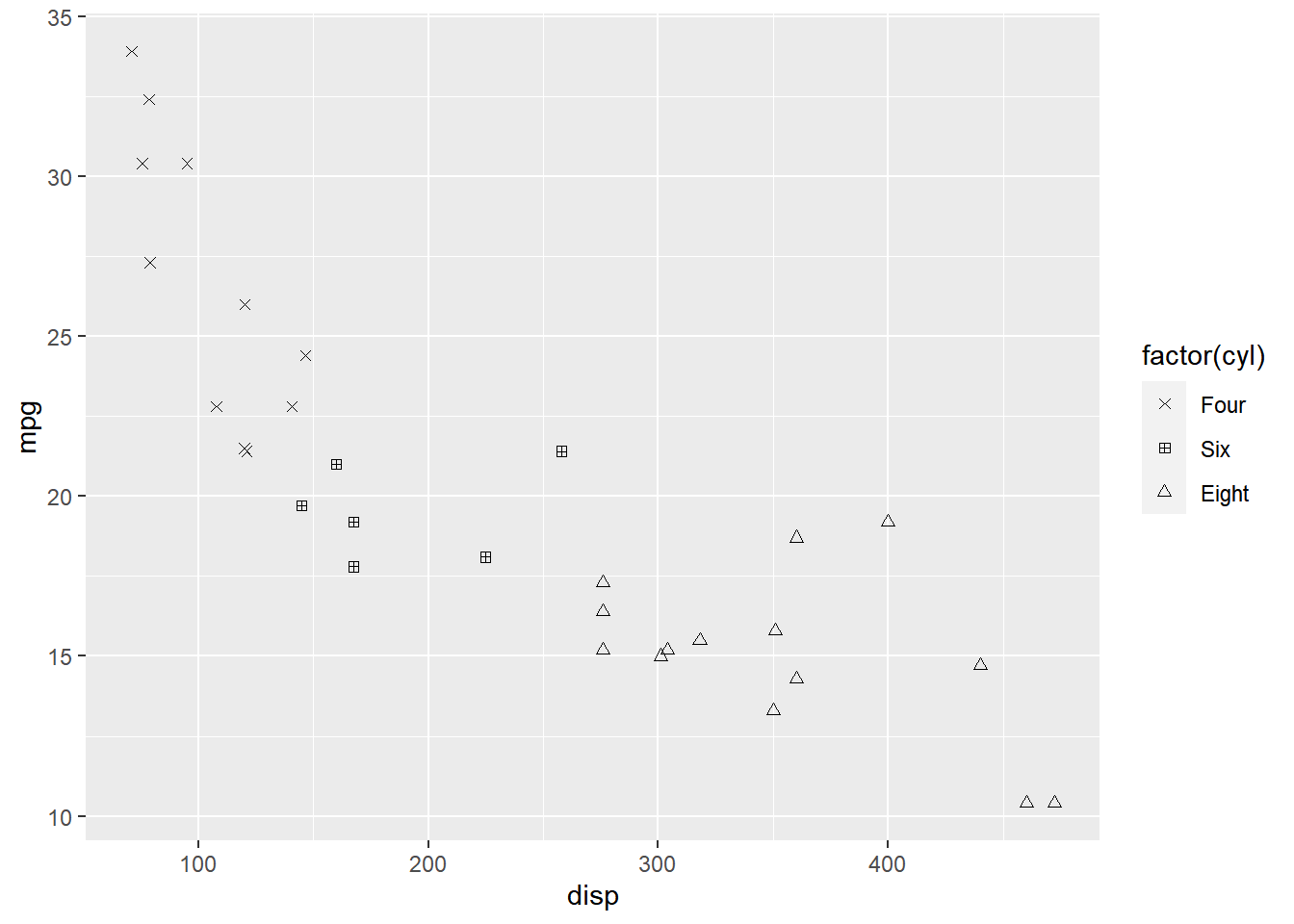### Limits

Let us assume that we want to modify the data to be displayed i.e. instead of examining the relationship between mileage and displacement for all cars, we desire to look at only cars with at least 6 cylinders. One way to approach this would be to filter the data using `filter` from dplyr and then visualize it. Instead, we will use the `limits` argument and filter the data for visualization.

``````ggplot(mtcars) +
geom_point(aes(disp, mpg, shape = factor(cyl))) +
scale_shape_manual(values = c(4, 24), limits = c(6, 8))``````
``````## Warning: Continuous limits supplied to discrete scale.
## Did you mean `limits = factor(...)` or `scale_*_continuous()`?``````
``## Warning: Removed 11 rows containing missing values (geom_point).``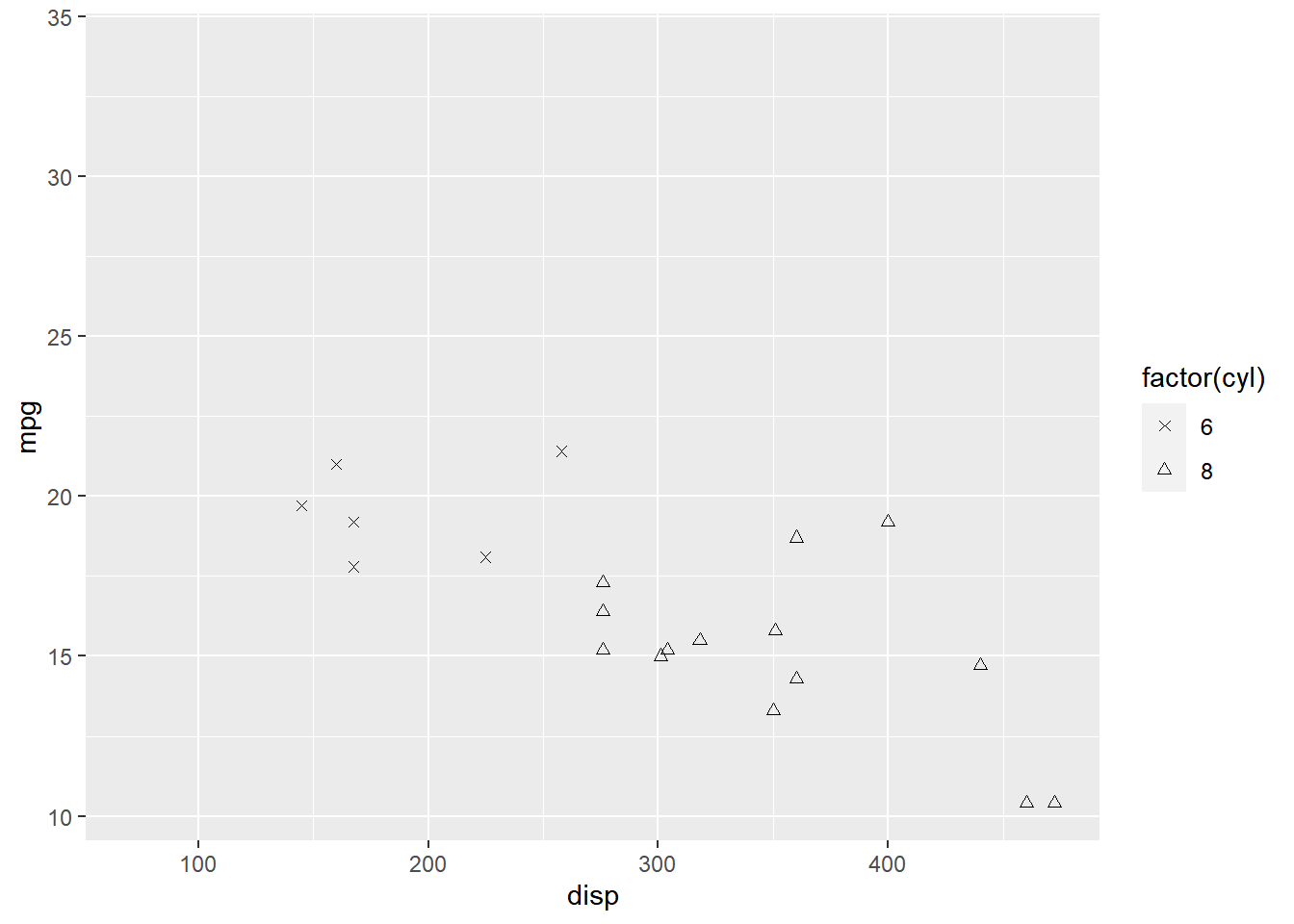As you can see above, `ggplot2` returns a warning message indicating data related to 4 cylinders has been dropped. If you observe the legend, it now represents only 4 and 6 cylinders.

### Breaks

When there are large number of levels in the mapped variable, you may not want the labels in the legend to represent all of them. In such cases, we can use the breaks argument and specify the labels to be used. In the below case, we use the `breaks` argument to ensure that the labels in legend represent two levels (4, 8) of the mapped variable.

``````ggplot(mtcars) +
geom_point(aes(disp, mpg, shape = factor(cyl))) +
scale_shape_manual(values = c(4, 12, 24), breaks = c(4, 8))``````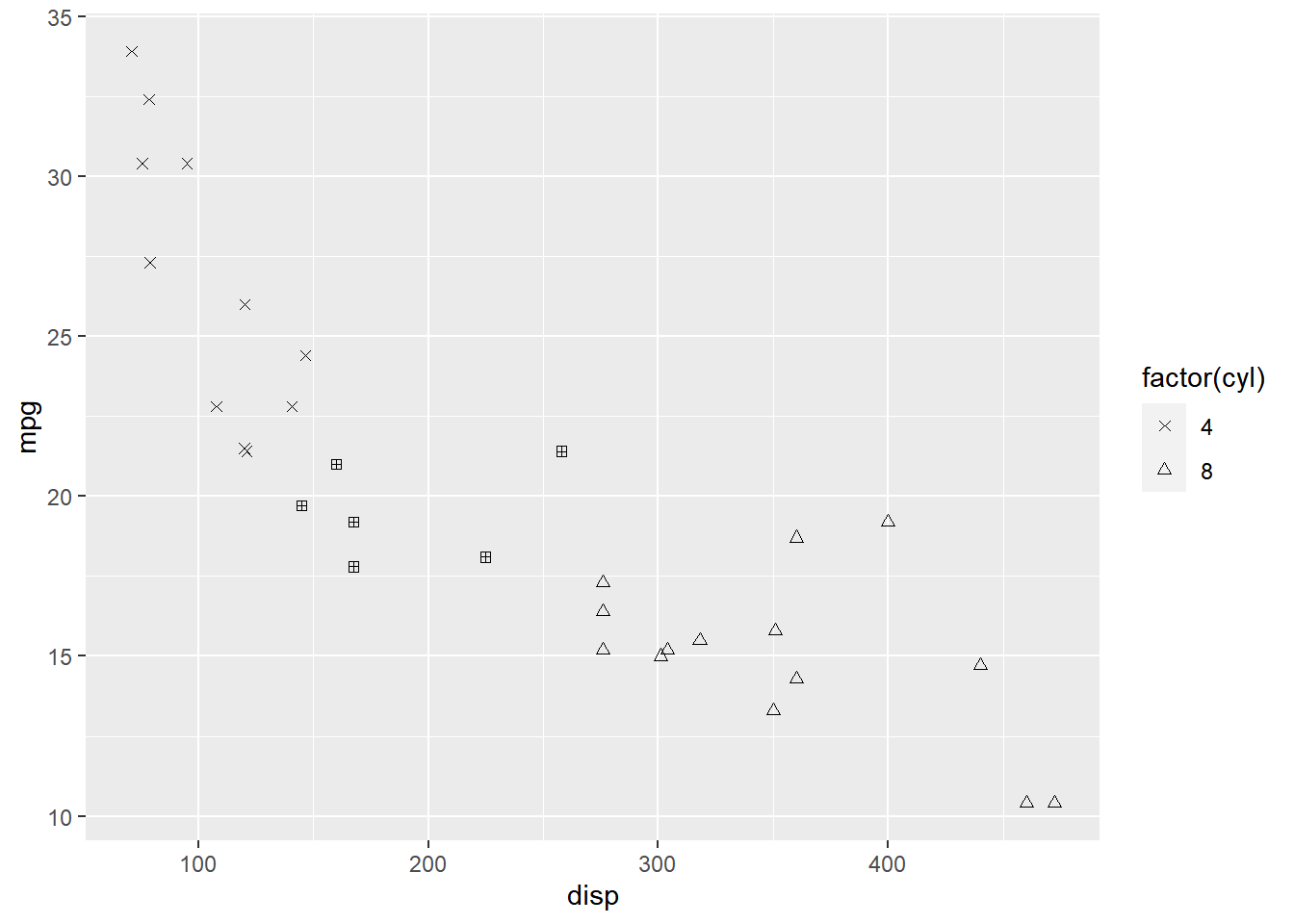### Putting it all together…

``````ggplot(mtcars) +
geom_point(aes(disp, mpg, shape = factor(cyl))) +
scale_shape_manual(name = "Cylinders", labels = c('Six', 'Eight'),
values = c(4, 24), limits = c(6, 8), breaks = c(6, 8))``````
``````## Warning: Continuous limits supplied to discrete scale.
## Did you mean `limits = factor(...)` or `scale_*_continuous()`?``````
``## Warning: Removed 11 rows containing missing values (geom_point).``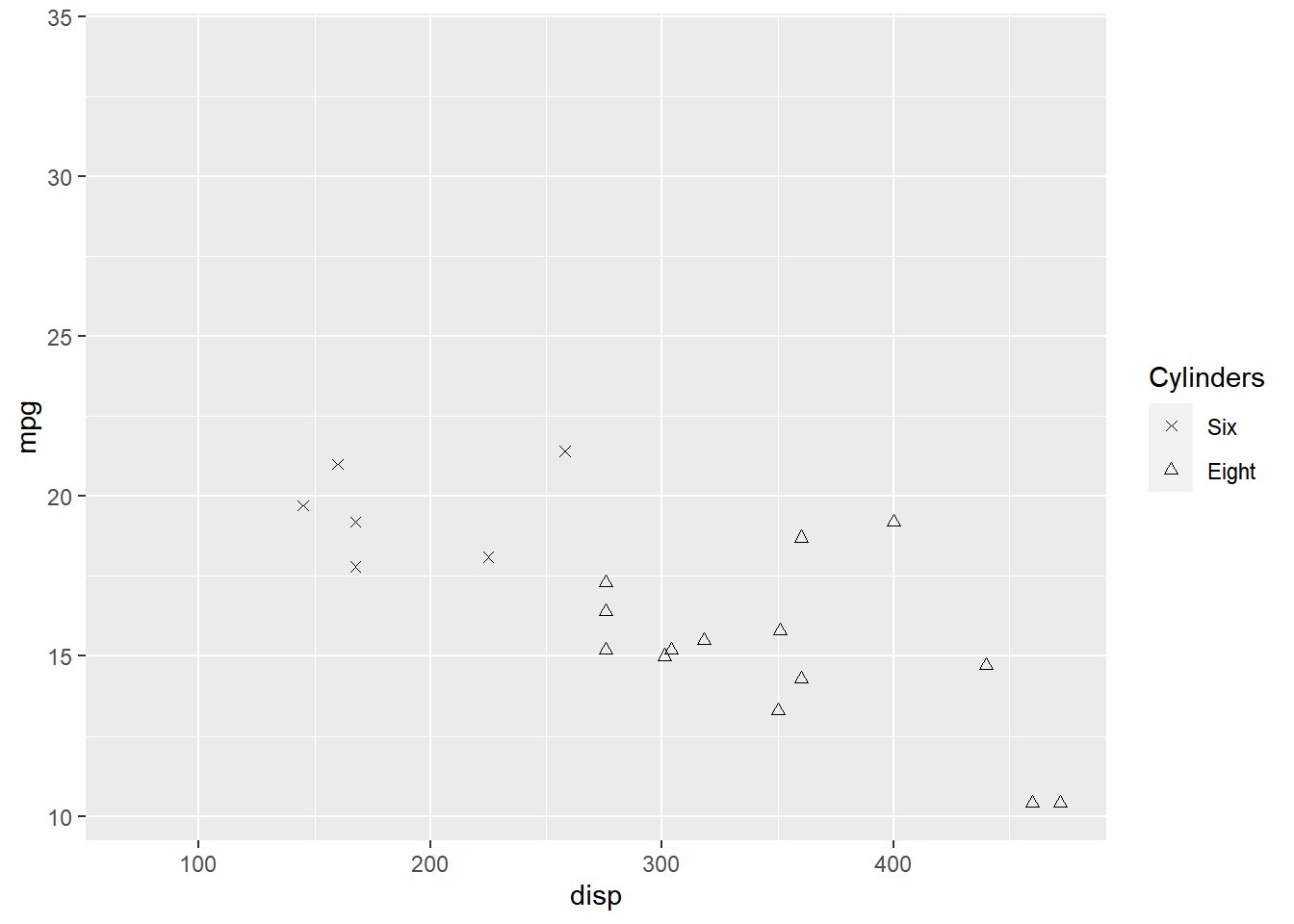## 12.4 Size

We will learn to modify the following using `scale_size_continuous` when `size` aesthetic is mapped to variables:

• title
• breaks
• limits
• range
• labels
• values

### Plot

Let us start with a scatter plot examining the relationship between displacement and miles per gallon from the mtcars data set. We will map the size of the points to the `hp` variable. Remember, `size` must always be mapped to a continuous variable.

``````ggplot(mtcars) +
geom_point(aes(disp, mpg, size = hp))``````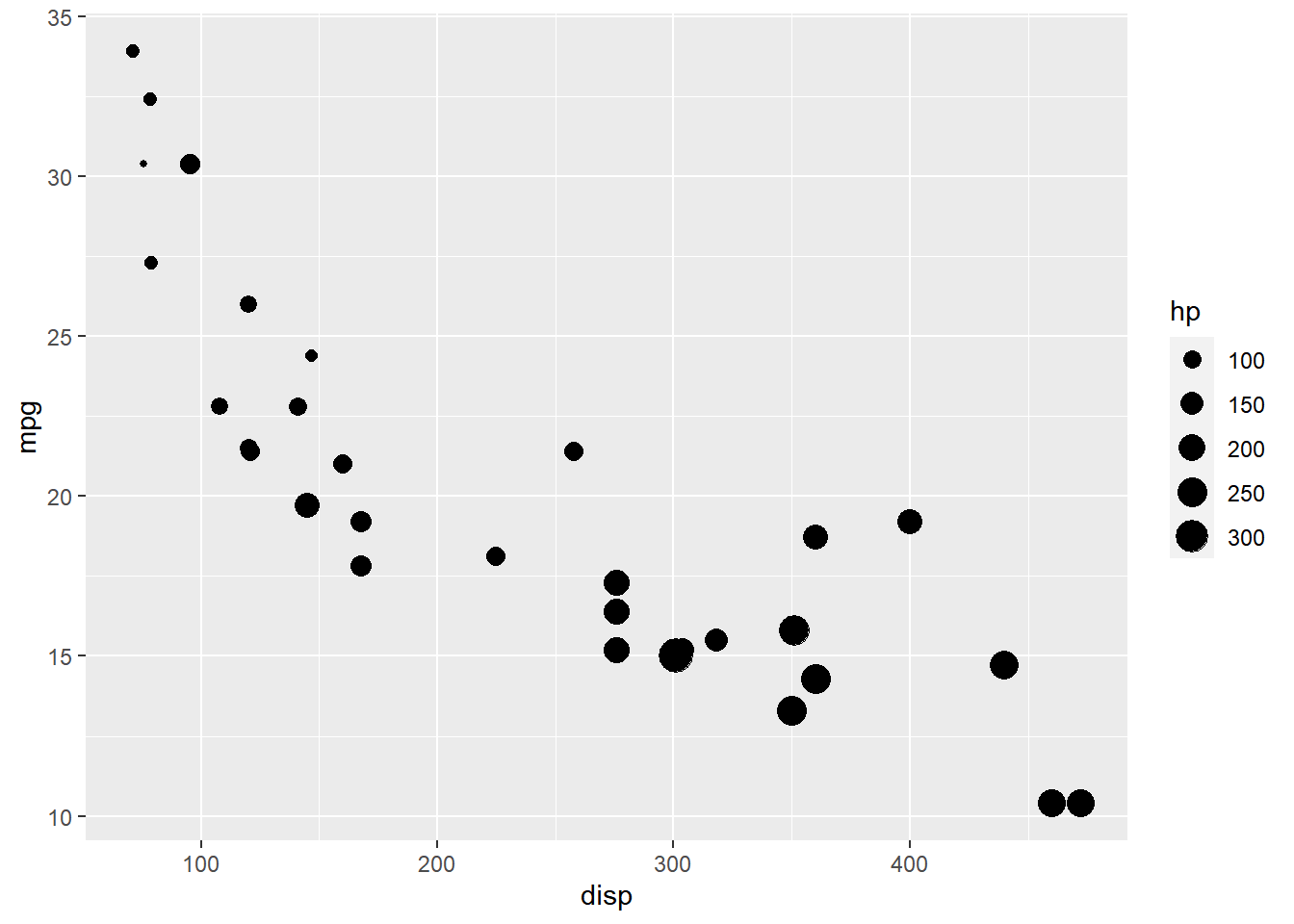As you can see, the legend acts as a guide for the `size` aesthetic. Now, let us learn to modify the different aspects of the legend.

### Title

The title of the legend (`hp`) is not very intuitive. If the user does not know the underlying data, they will not be able to make any sense out of it. Let us change it to `Horsepower` using the `name` argument.

``````ggplot(mtcars) +
geom_point(aes(disp, mpg, size = hp)) +
scale_size_continuous(name = "Horsepower")``````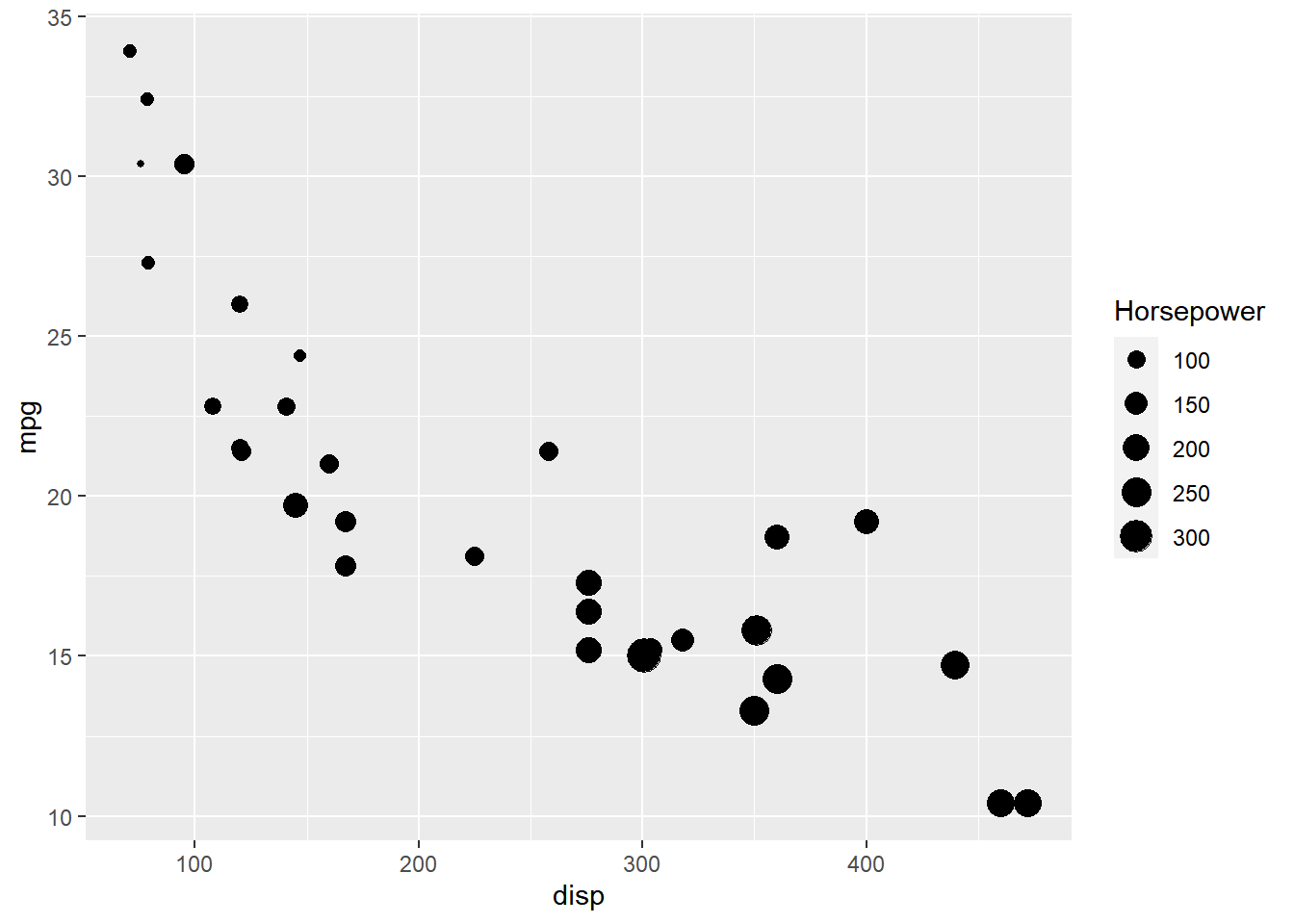### Range

The range of the size of points can be modified using the `range` argument. We need to specify a lower and upper range using a numeric vector. In the below example, we use `range` and supply the lower and upper limits as `3` and `6`. The size of the points will now lie between `3` and `6` only.

``````ggplot(mtcars) +
geom_point(aes(disp, mpg, size = hp)) +
scale_size_continuous(range = c(3, 6))``````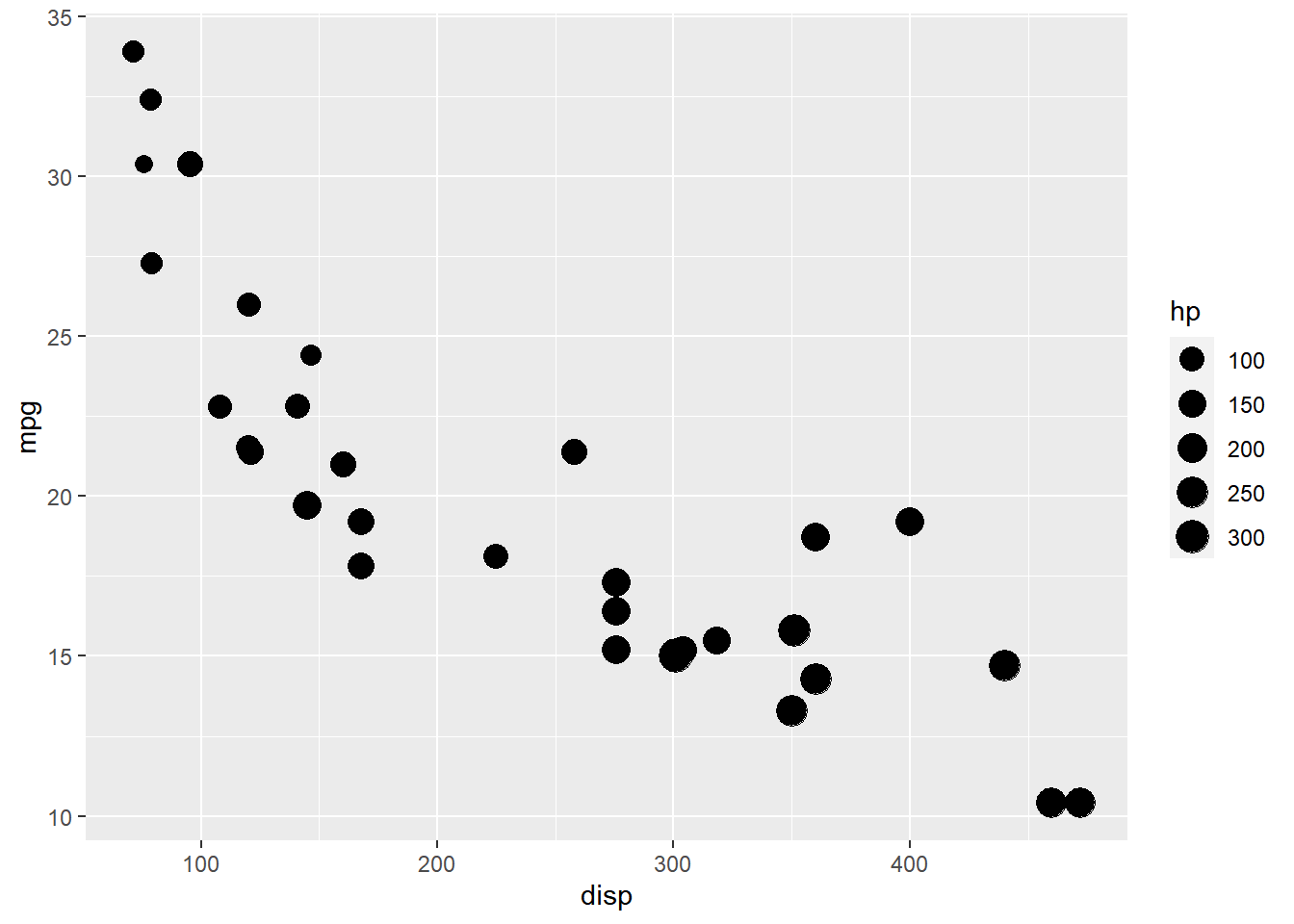### Limits

Let us assume that we want to modify the data to be displayed i.e. instead of examining the relationship between mileage and displacement for all cars, we desire to look at only cars whose horsepower is between `100` and `350`. One way to approach this would be to filter the data using `filter` from dplyr and then visualize it. Instead, we will use the `limits` argument and filter the data for visualization.

``````ggplot(mtcars) +
geom_point(aes(disp, mpg, size = hp)) +
scale_size_continuous(limits = c(100, 350))``````
``## Warning: Removed 9 rows containing missing values (geom_point).``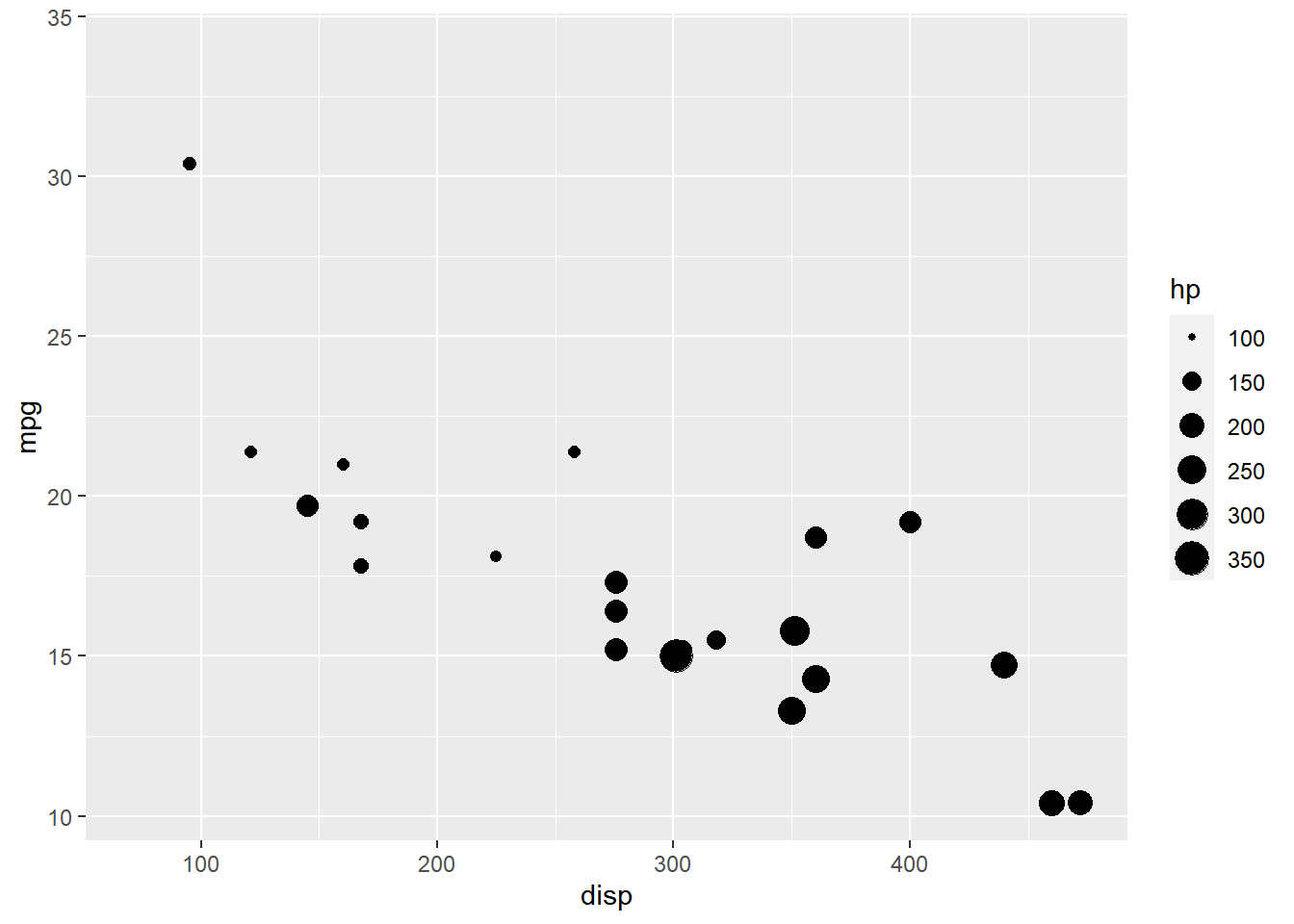### Breaks

When the range of the variable mapped to size is large, you may not want the labels in the legend to represent all of them. In such cases, we can use the breaks argument and specify the labels to be used. In the below case, we use the `breaks` argument to ensure that the labels in legend represent certain midpoints (125, 200, 275) of the mapped variable.

``````ggplot(mtcars) +
geom_point(aes(disp, mpg, size = hp)) +
scale_size_continuous(breaks = c(125, 200, 275))``````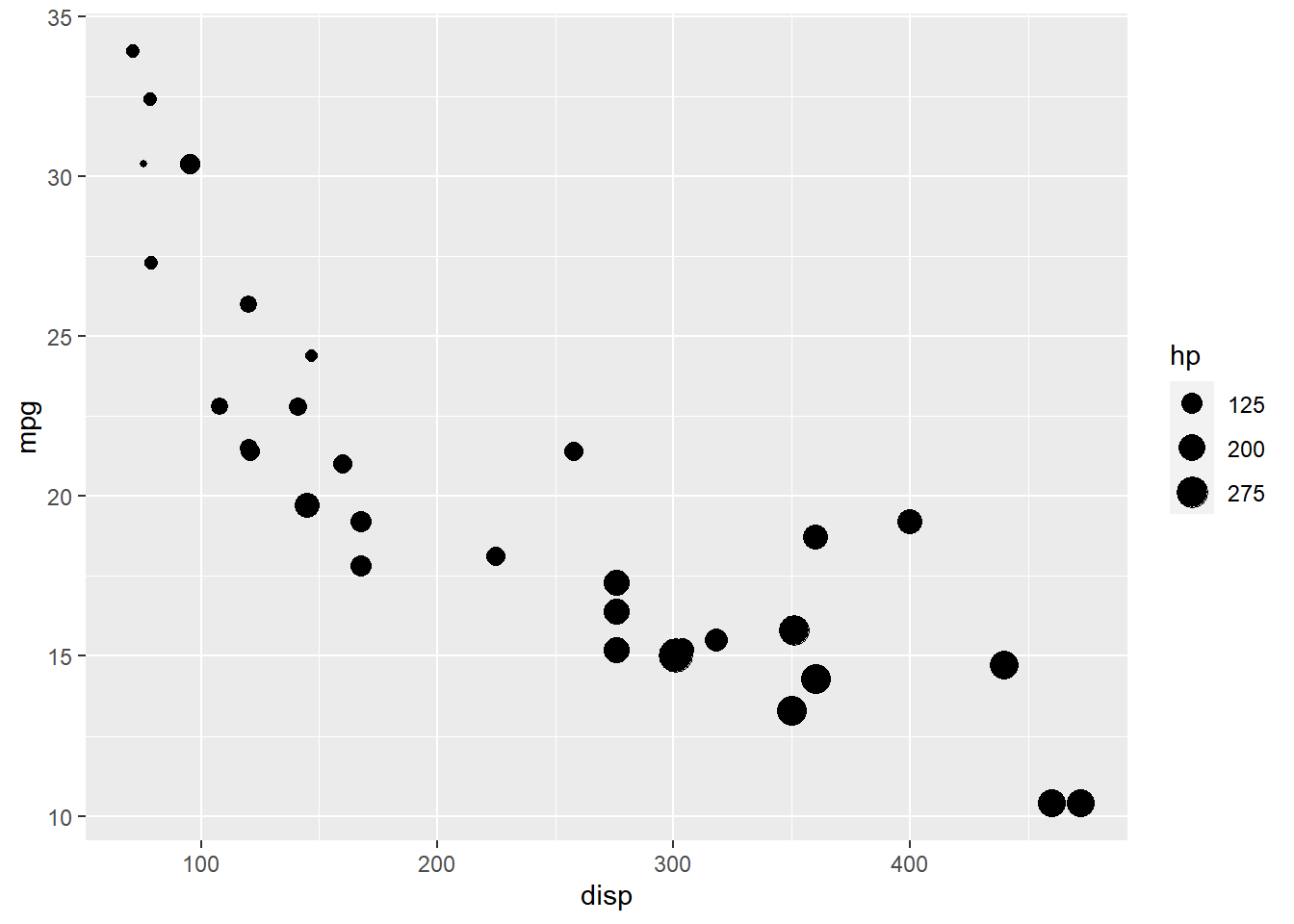### Labels

The labels in the legend can be modified using the `labels` argument. Let us change the labels to “1 Hundred”, “2 Hundred” and “3 Hundred” in the next example. Ensure that the labels are intuitive and easy to interpret for the end user of the plot.

``````ggplot(mtcars) +
geom_point(aes(disp, mpg, size = hp)) +
scale_size_continuous(breaks = c(100, 200, 300),
labels = c("1 Hundred", "2 Hundred", "3 Hundred"))``````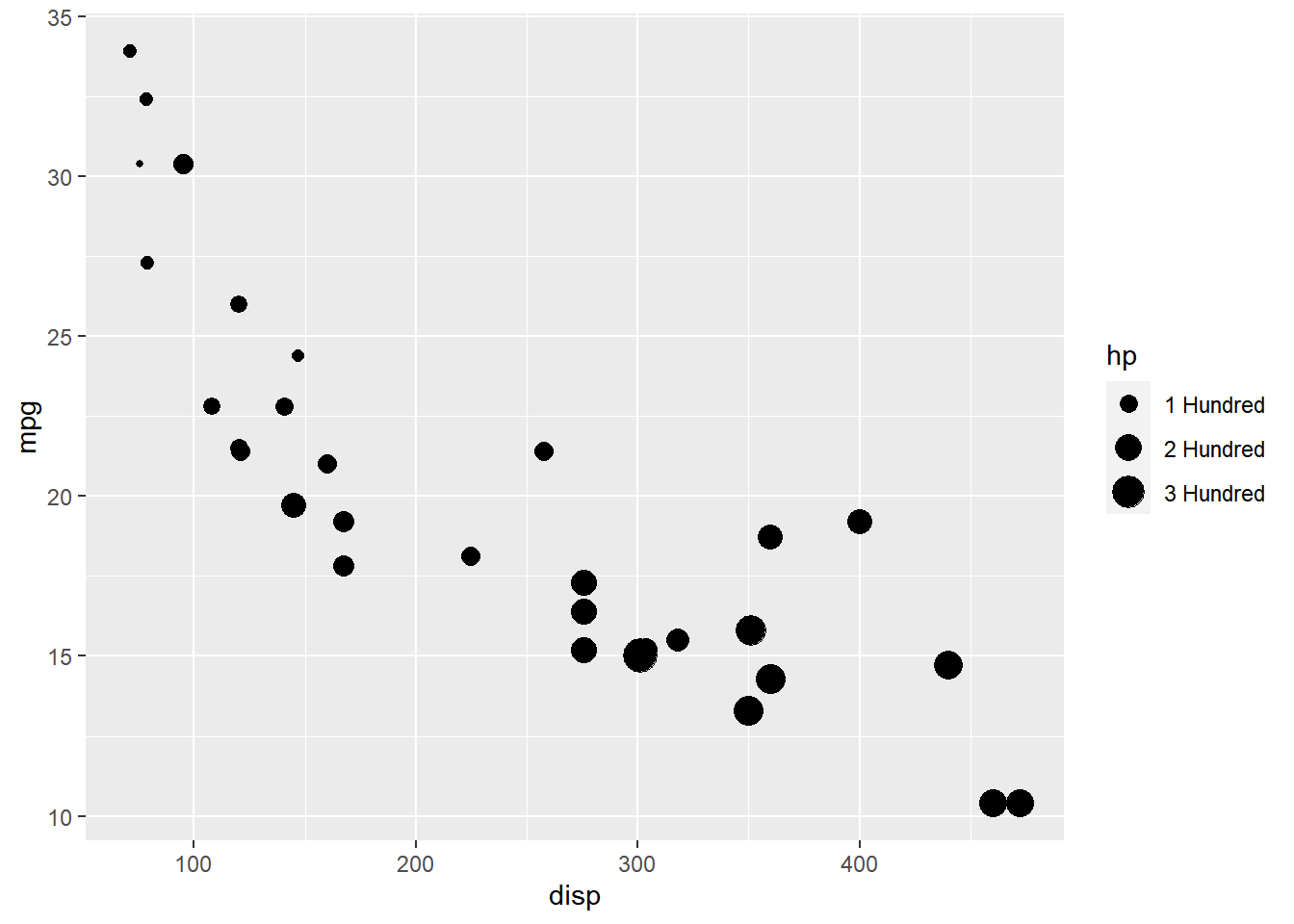### Putting it all together…

``````ggplot(mtcars) +
geom_point(aes(disp, mpg, size = hp)) +
scale_size_continuous(name = "Horsepower", range = c(3, 6),
limits = c(0, 400), breaks = c(100, 200, 300),
labels = c("1 Hundred", "2 Hundred", "3 Hundred"))``````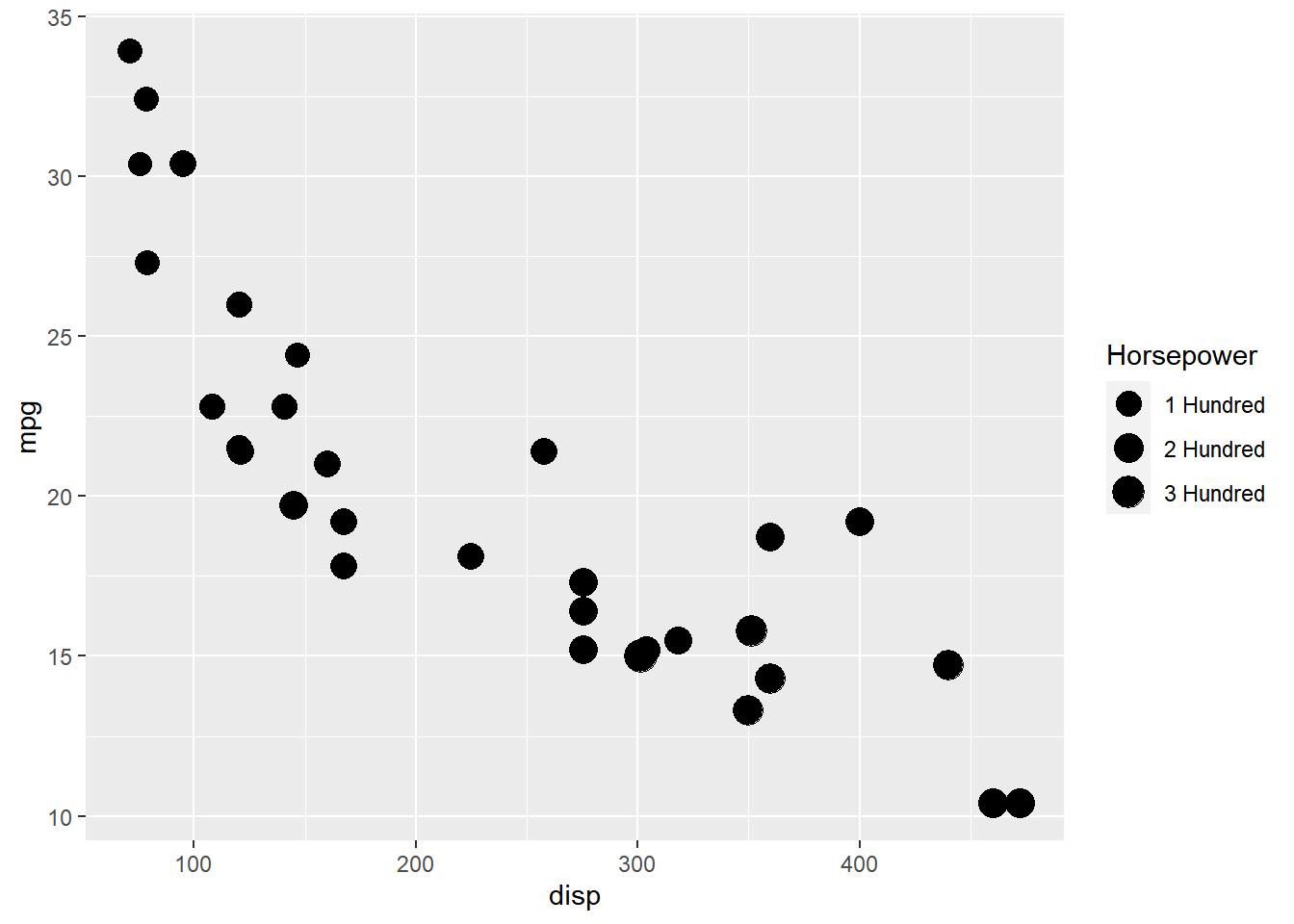## 12.5 Transparency

We will learn to modify the following using `scale_alpha_continuous()` when `alpha` or transparency is mapped to variables:

• title
• breaks
• limits
• range
• labels
• values

### Plot

Let us start with a scatter plot examining the relationship between displacement and miles per gallon from the mtcars data set. We will map the transparency of the points to the `hp` variable. Remember, `alpha` must always be mapped to a continuous variable.

``````ggplot(mtcars) +
geom_point(aes(disp, mpg, alpha = hp), color = 'blue')``````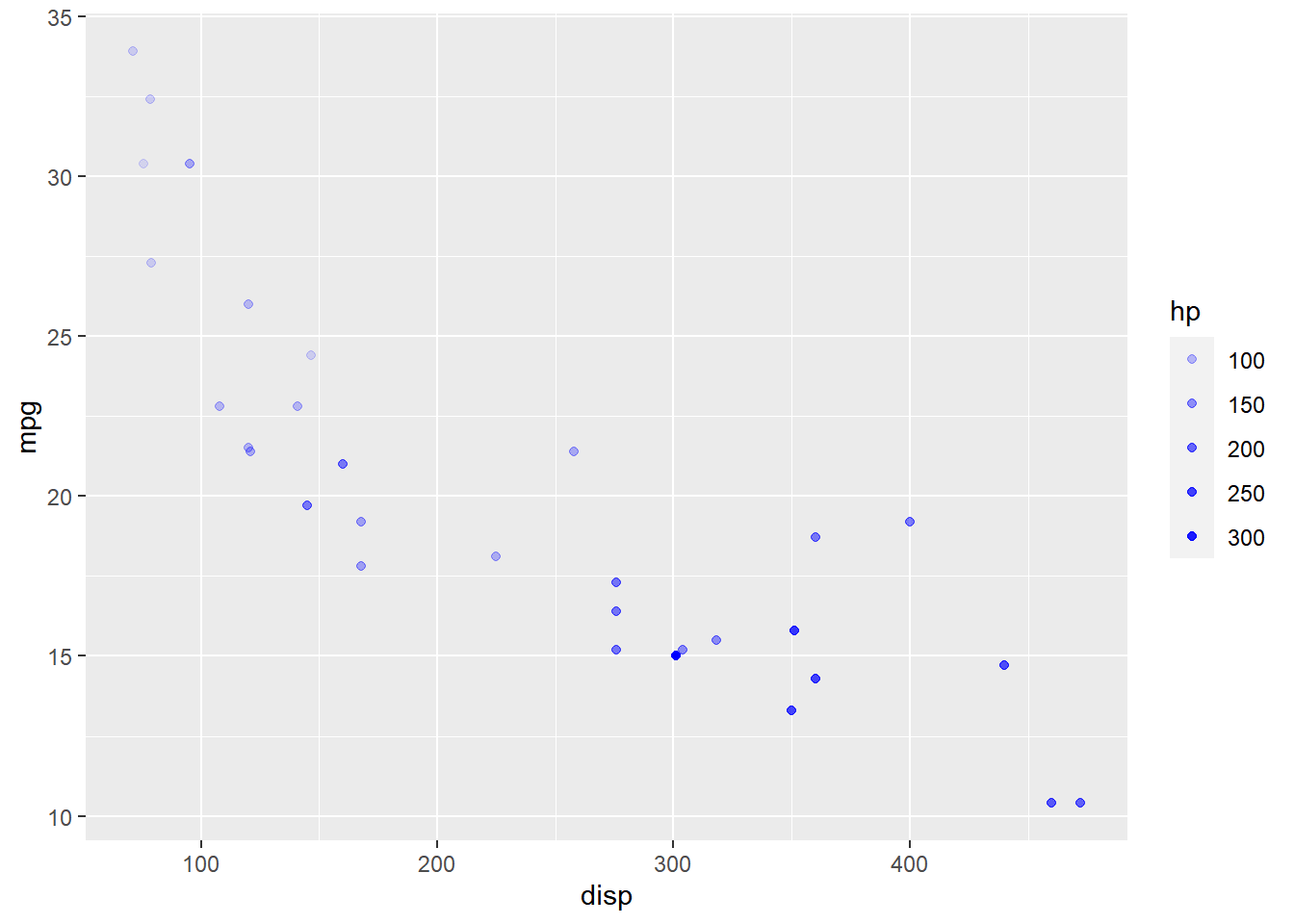As you can see, the legend acts as a guide for the `alpha` aesthetic. Now, let us learn to modify the different aspects of the legend.

### Title

The title of the legend (`hp`) is not very intuitive. If the user does not know the underlying data, they will not be able to make any sense out of it. Let us change it to `Horsepower` using the `name` argument.

``````ggplot(mtcars) +
geom_point(aes(disp, mpg, alpha = hp), color = 'blue') +
scale_alpha_continuous("Horsepower")``````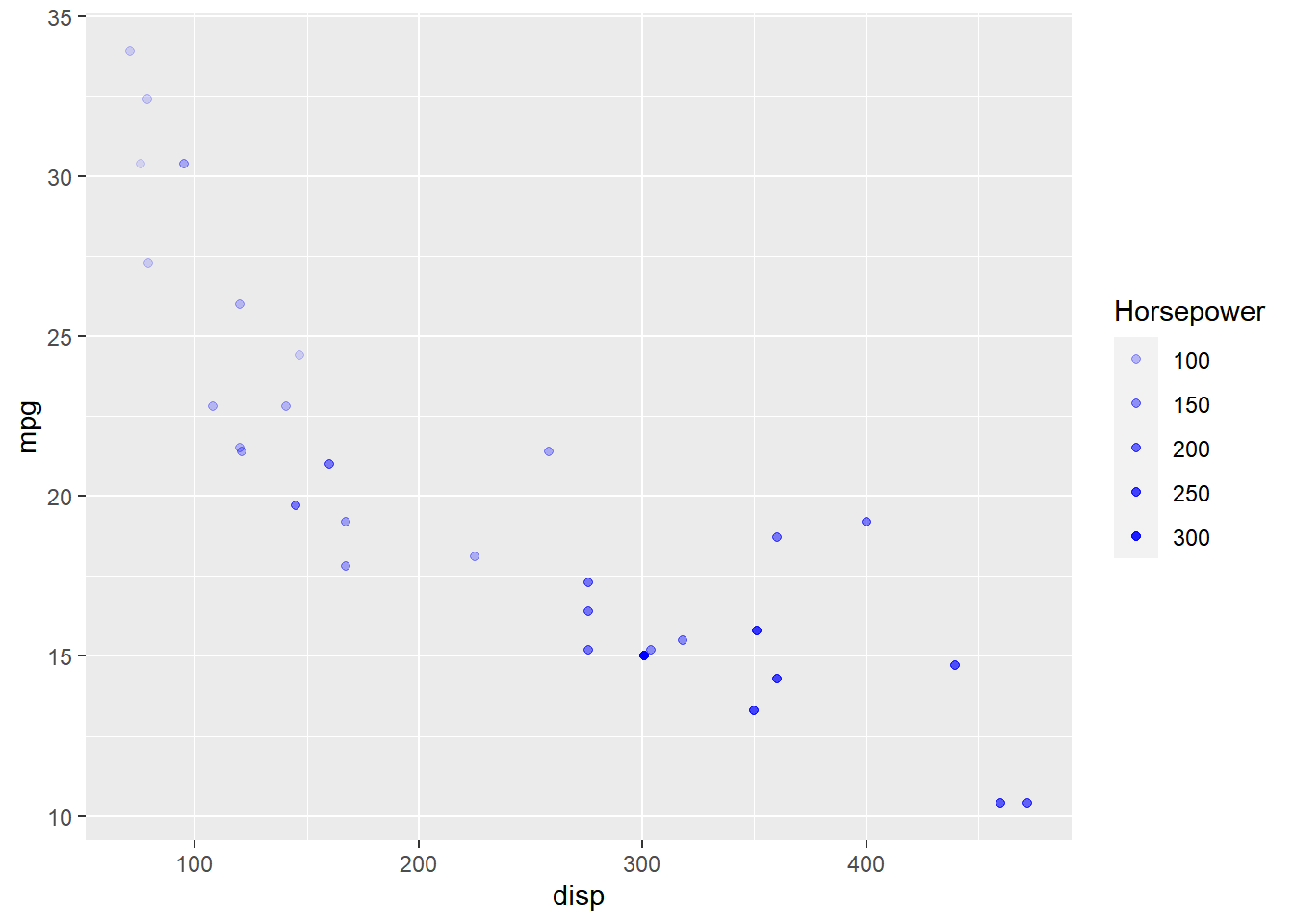### Breaks

When the range of the variable mapped to size is large, you may not want the labels in the legend to represent all of them. In such cases, we can use the breaks argument and specify the labels to be used. In the below case, we use the `breaks` argument to ensure that the labels in legend represent certain midpoints (125, 200, 275) of the mapped variable.

``````ggplot(mtcars) +
geom_point(aes(disp, mpg, alpha = hp), color = 'blue') +
scale_alpha_continuous(breaks = c(125, 200, 275))``````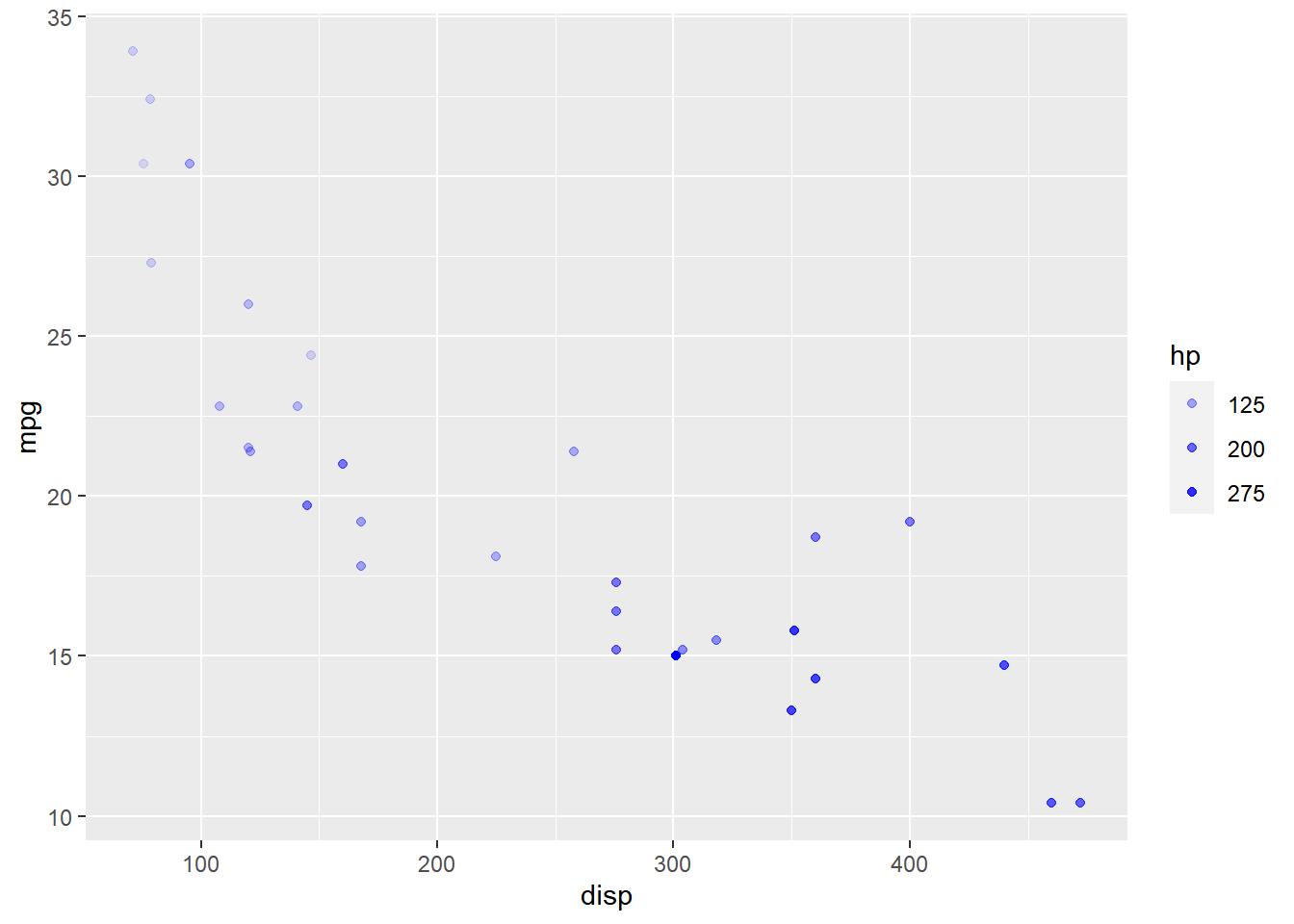### Limits

Let us assume that we want to modify the data to be displayed i.e. instead of examining the relationship between mileage and displacement for all cars, we desire to look at only cars whose horsepower is between `100` and `350`. One way to approach this would be to filter the data using `filter` from dplyr and then visualize it. Instead, we will use the `limits` argument and filter the data for visualization.

``````ggplot(mtcars) +
geom_point(aes(disp, mpg, alpha = hp), color = 'blue') +
scale_alpha_continuous(limits = c(100, 350))``````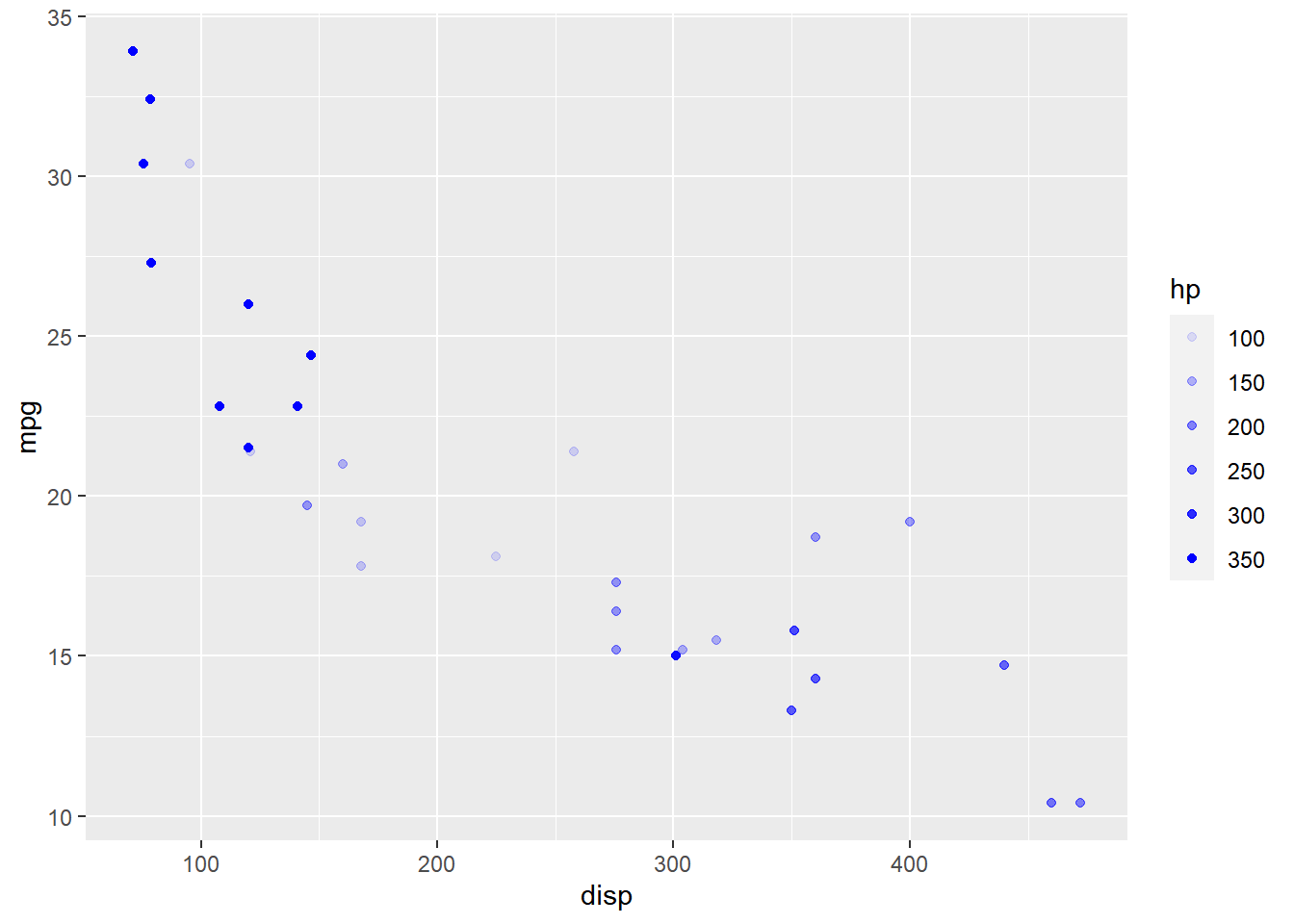### Range

The range of the transparency of points can be modified using the `range` argument. We need to specify a lower and upper range using a numeric vector. In the below example, we use `range` and supply the lower and upper limits as `0.4` and `0.8`. The transparency of the points will now lie between `0.4` and `0.8` only.

``````ggplot(mtcars) +
geom_point(aes(disp, mpg, alpha = hp), color = 'blue') +
scale_alpha_continuous(range = c(0.4, 0.8))``````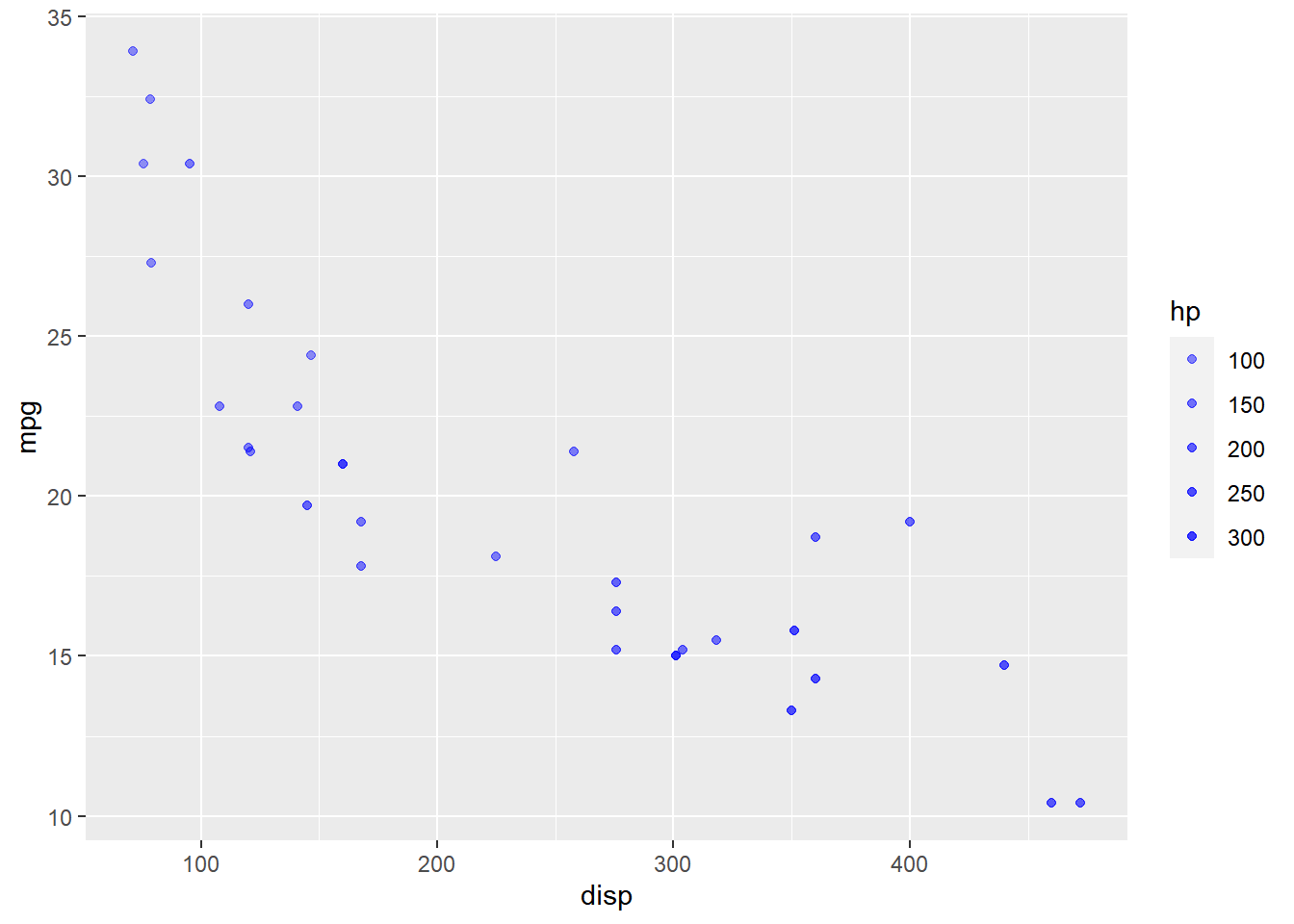### Labels

The labels in the legend can be modified using the `labels` argument. Let us change the labels to “1 Hundred”, “2 Hundred” and “3 Hundred” in the next example. Ensure that the labels are intuitive and easy to interpret for the end user of the plot.

``````ggplot(mtcars) +
geom_point(aes(disp, mpg, alpha = hp), color = 'blue') +
scale_alpha_continuous(breaks = c(100, 200, 300),
labels = c("1 Hundred", "2 Hundred",
"3 Hundred"))``````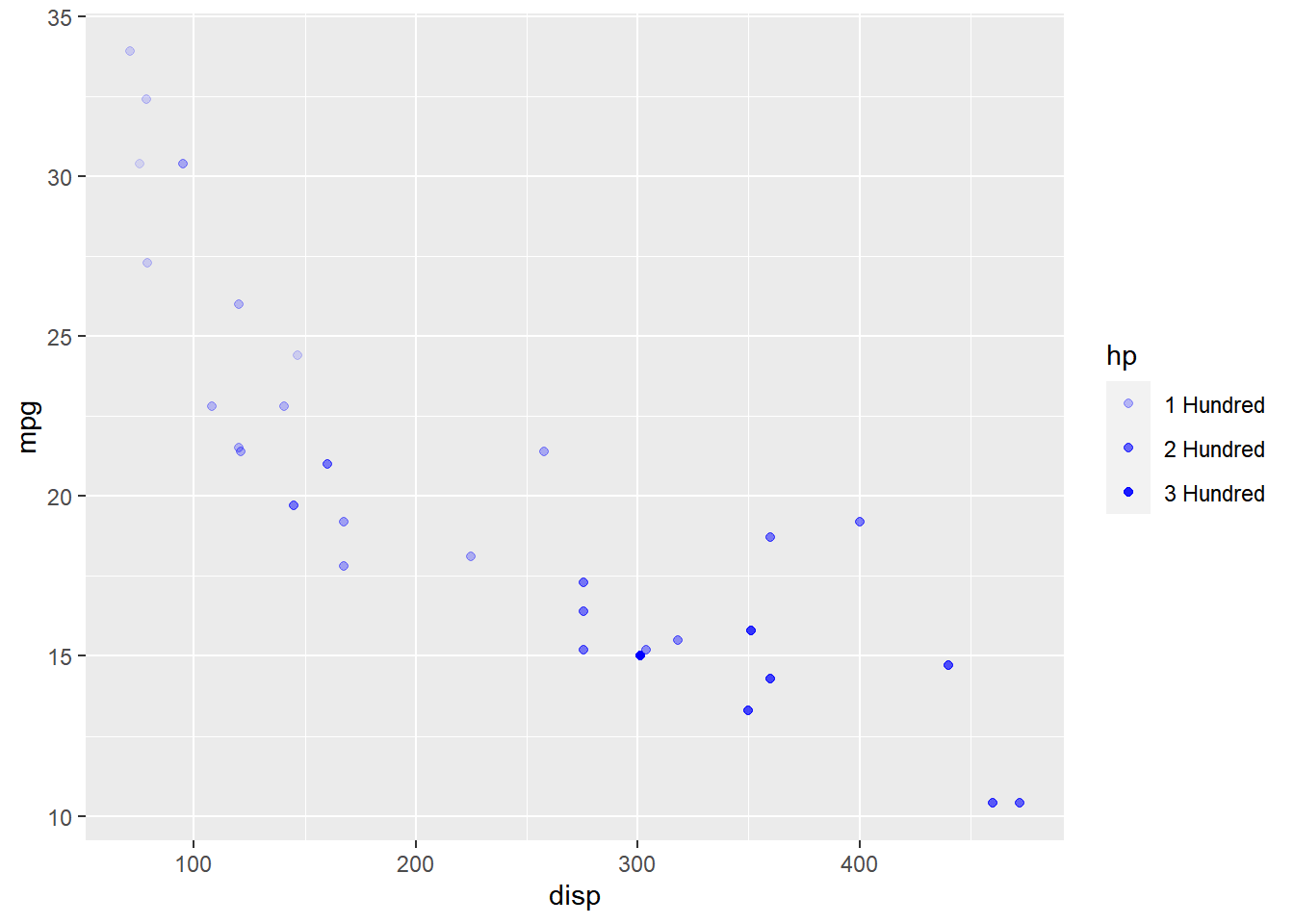### Putting it all together…

``````ggplot(mtcars) +
geom_point(aes(disp, mpg, alpha = hp), color = 'blue') +
scale_alpha_continuous("Horsepower", breaks = c(100, 200, 300),
limits = c(100, 350), range = c(0.4, 0.8),
labels = c("1 Hundred", "2 Hundred", "3 Hundred"))``````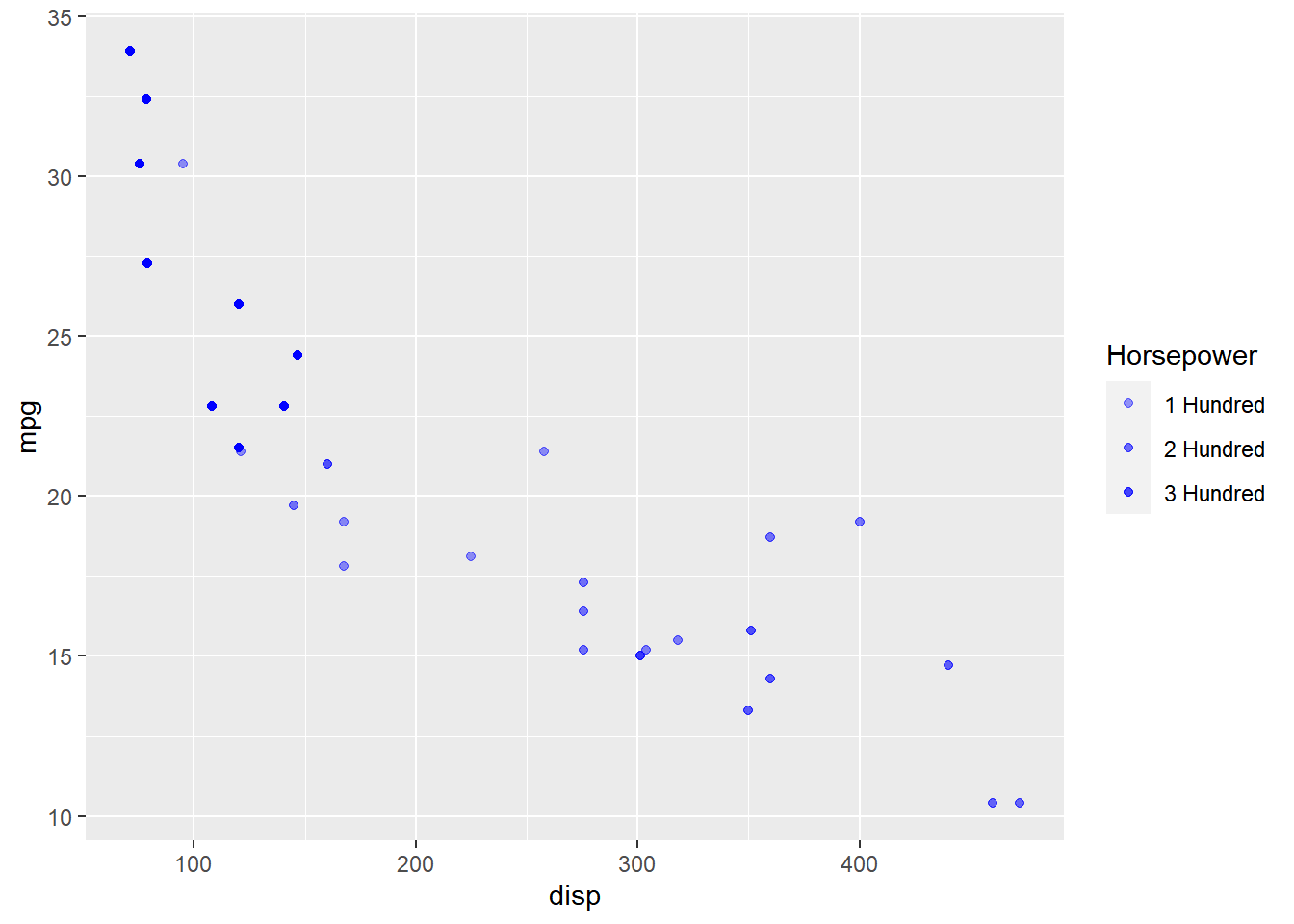## 12.6 Guide

In this section, we will learn to modify

• title
• label
• and bar

So far, we have learnt to modify the components of a legend using `scale_*` family of functions. Now, we will use the `guide` argument and supply it values using the `guide_legend()` function.

### Title

#### Title Alignment

The horizontal alignment of the title can be managed using the `title.hjust` argument. It can take any value between `0` and `1`.

• 0 (left)
• 1 (right)

In the below example, we align the title to the center by assigning the value `0.5`.

``````ggplot(mtcars) + geom_point(aes(disp, mpg, color = factor(cyl))) +
scale_color_manual(values = c("red", "blue", "green"),
guide = guide_legend(title = "Cylinders", title.hjust = 0.5))``````#### Title Alignment (Vertical)

To manage the vertical alignment of the title, use `title.vjust`.

``````ggplot(mtcars) + geom_point(aes(disp, mpg, color = hp)) +
scale_color_continuous(guide = guide_colorbar(
title = "Horsepower", title.position = "top", title.vjust = 1))``````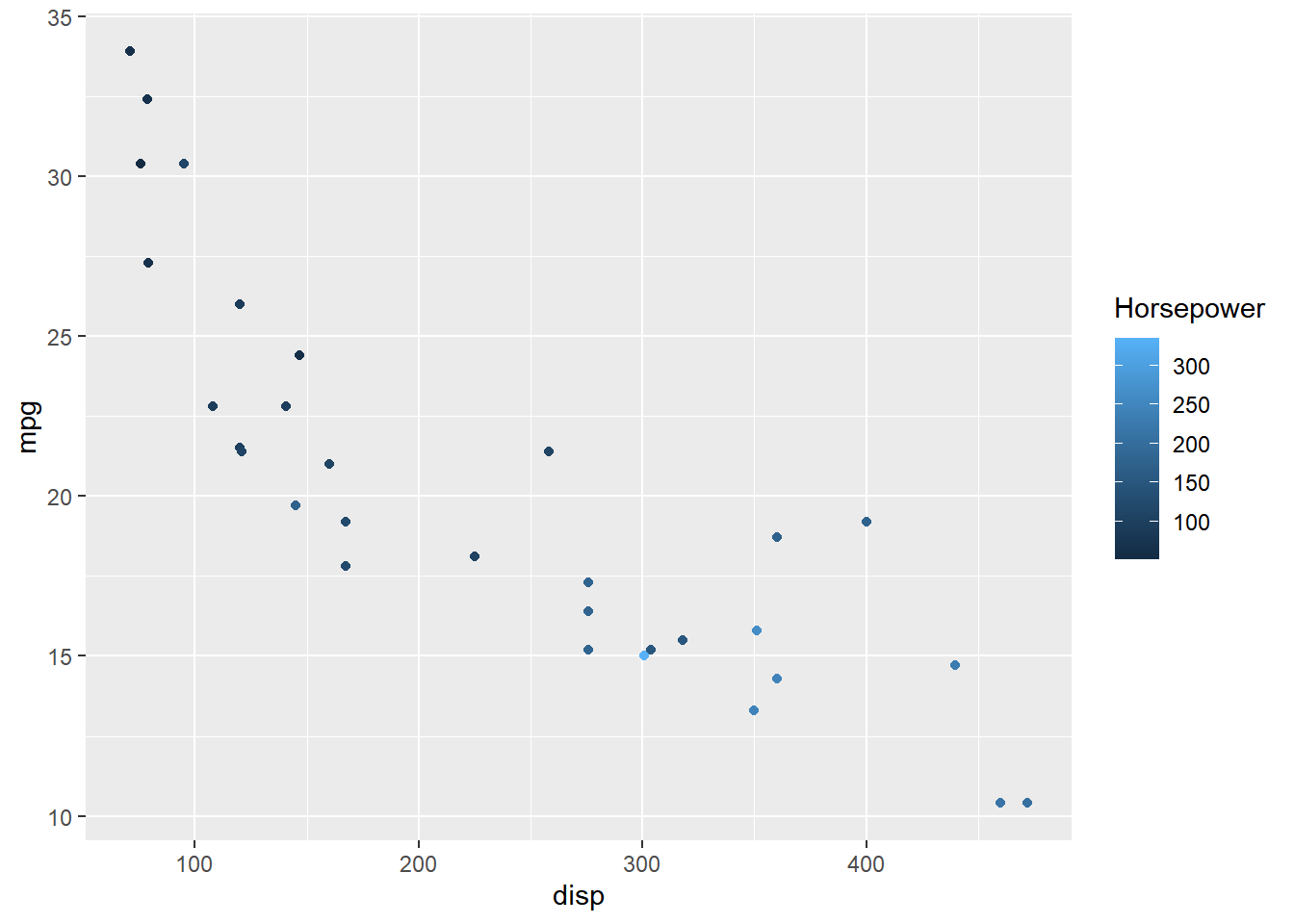#### Title Position

The position of the title can be managed using `title.posiiton` argument. It can be positioned at:

• top
• bottom
• left
• right
``````ggplot(mtcars) + geom_point(aes(disp, mpg, color = factor(cyl))) +
scale_color_manual(values = c("red", "blue", "green"),
guide = guide_legend(title = "Cylinders", title.hjust = 0.5,
title.position = "top"))``````### Label

#### Label Position

The position of the label can be managed using the `label.position` argument. It can be positioned at:

• top
• bottom
• left
• right

In the below example, we position the label at right.

``````ggplot(mtcars) + geom_point(aes(disp, mpg, color = factor(cyl))) +
scale_color_manual(values = c("red", "blue", "green"),
guide = guide_legend(label.position = "right"))``````#### Label Alignment

The horizontal alignment of the label can be managed using the `label.hjust` argument. It can take any value between `0` and `1`.

• 0 (left)
• 1 (right)

In the below example, we align the label to the center by assigning the value `0.5`.

• alignment
• 0 (left)
• 1 (right)
``````ggplot(mtcars) + geom_point(aes(disp, mpg, color = factor(cyl))) +
scale_color_manual(values = c("red", "blue", "green"),
guide = guide_legend(label.hjust = 0.5))``````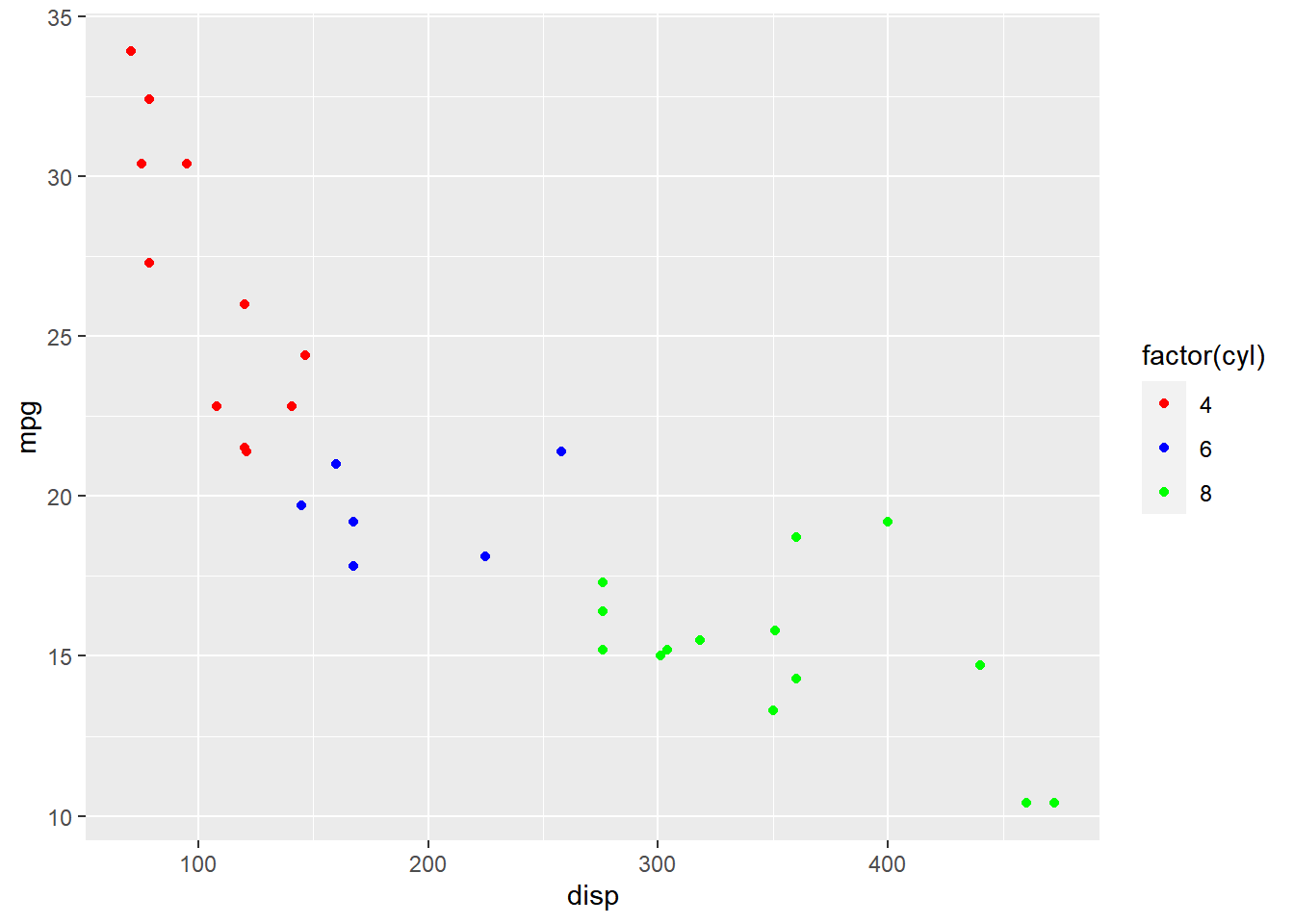#### Labels Alignment (Vertical)

The vertical alignment of the label can be managed using the `label.vjust` argument.

``````ggplot(mtcars) +
geom_point(aes(disp, mpg, color = hp)) +
scale_color_continuous(guide = guide_colorbar(
label.vjust = 0.8))``````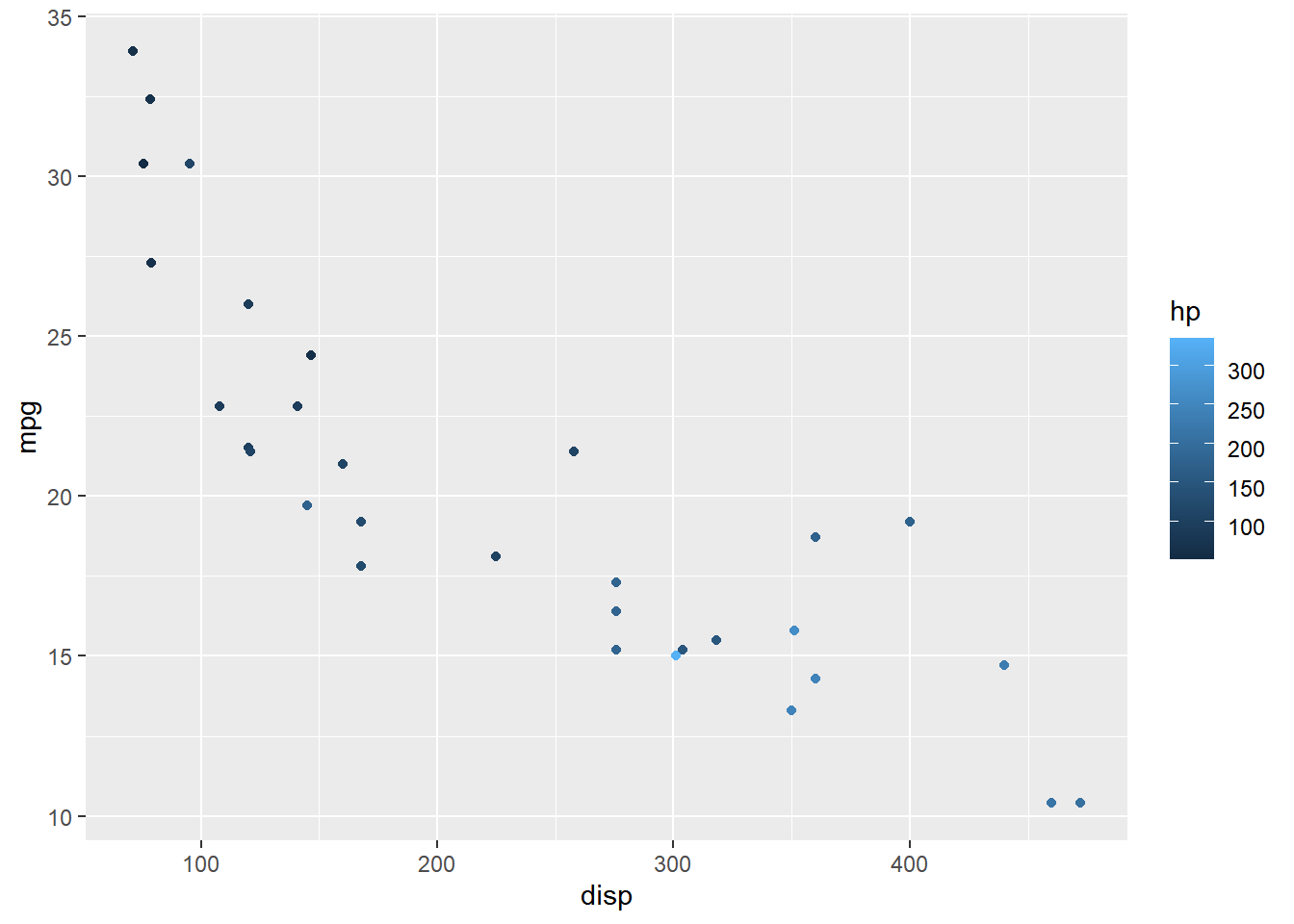#### Direction

The direction of the label can be either horizontal or veritcal and it can be set using the `direction` argument.

``````ggplot(mtcars) + geom_point(aes(disp, mpg, color = factor(cyl))) +
scale_color_manual(values = c("red", "blue", "green"),
guide = guide_legend(direction = "horizontal"))``````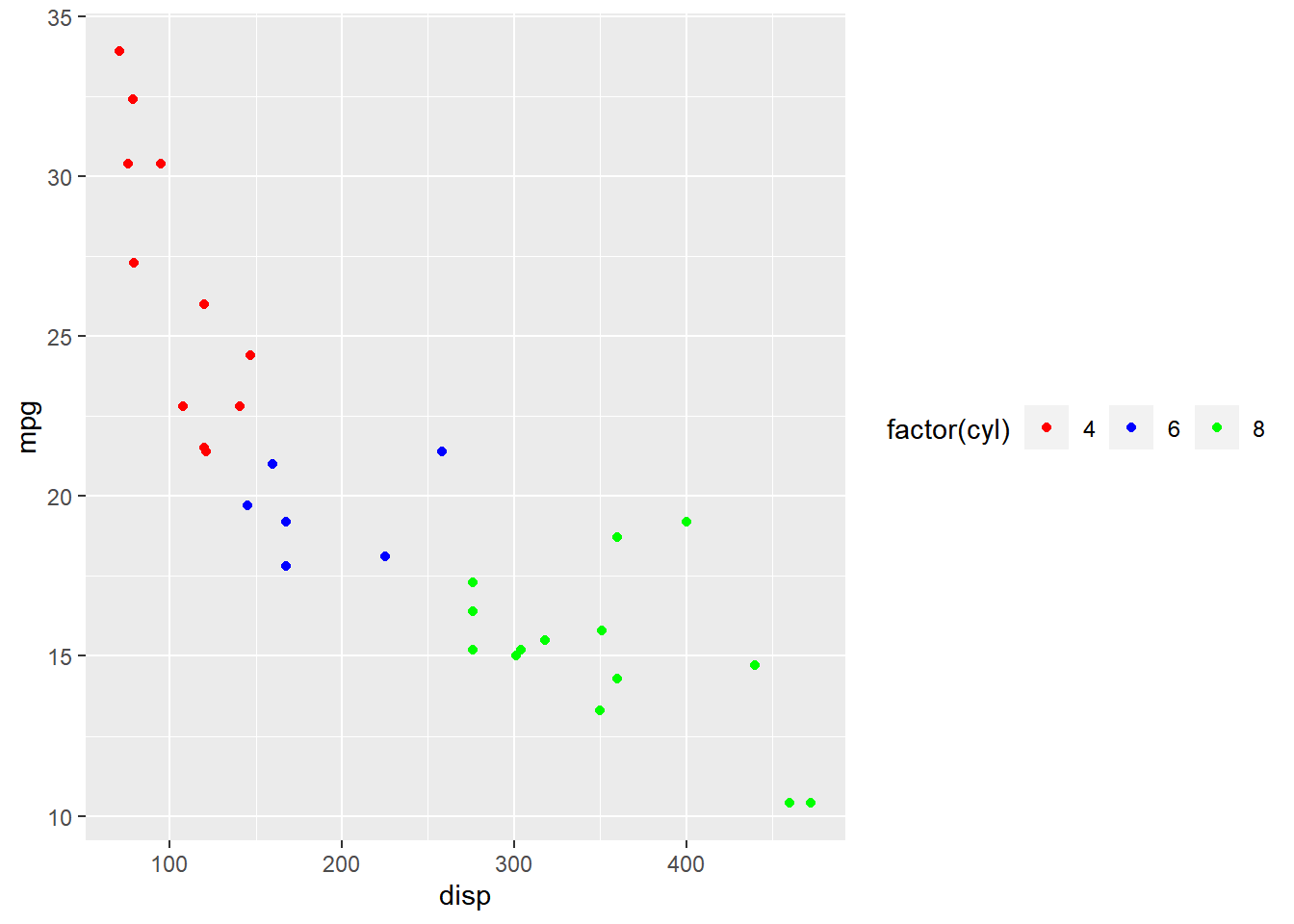#### Rows

The label can be spread across multiple rows using the `nrow` argument. In the below example, the label is spread across 2 rows.

``````ggplot(mtcars) + geom_point(aes(disp, mpg, color = factor(cyl))) +
scale_color_manual(values = c("red", "blue", "green"),
guide = guide_legend(nrow = 2))``````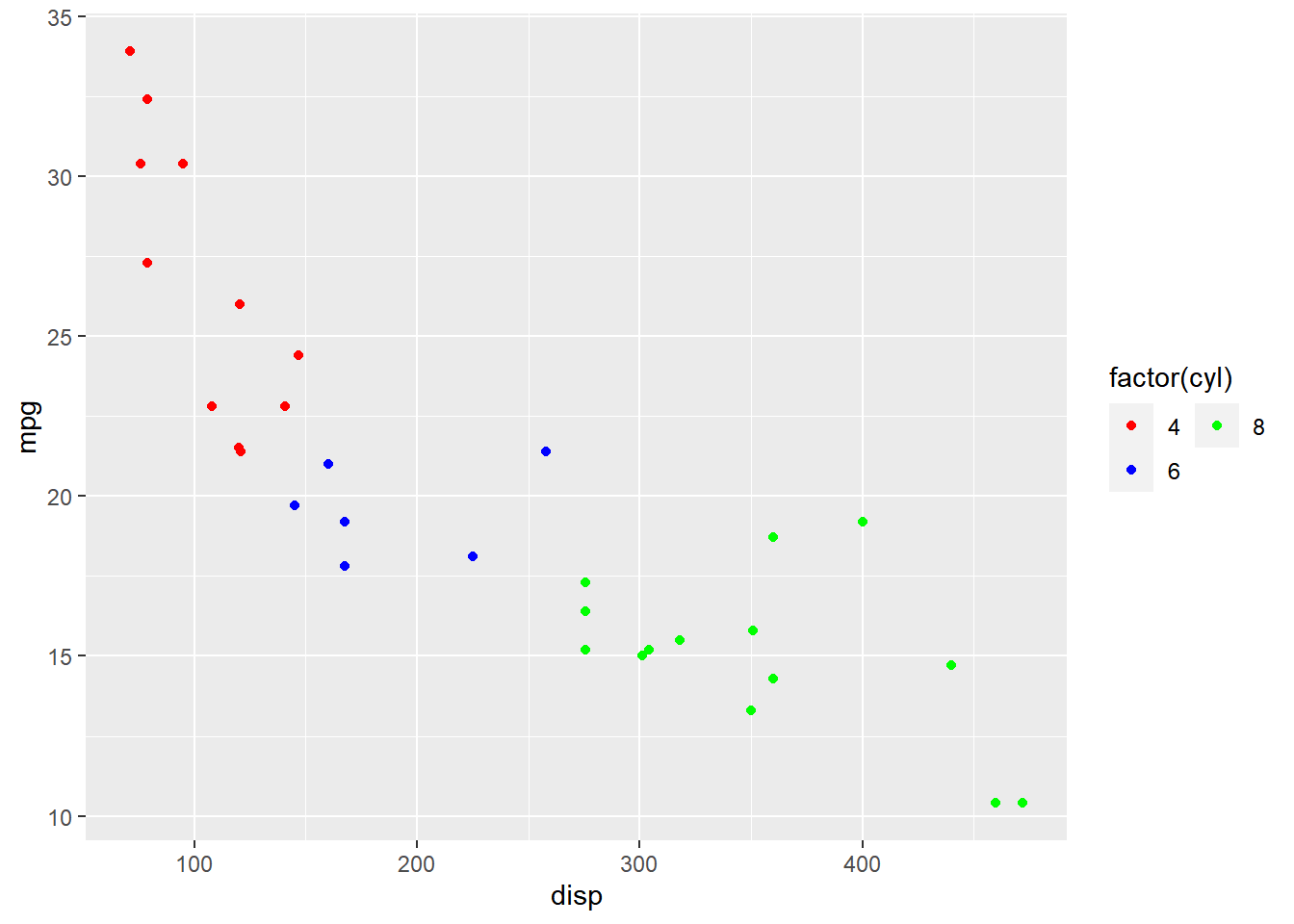#### Reverse

The order of the labels can be reversed using the `reverse` argument. We need to supply logical values i.e. either `TRUE` or `FALSE`. If `TRUE`, the order will be reversed.

``````ggplot(mtcars) + geom_point(aes(disp, mpg, color = factor(cyl))) +
scale_color_manual(values = c("red", "blue", "green"),
guide = guide_legend(reverse = TRUE))``````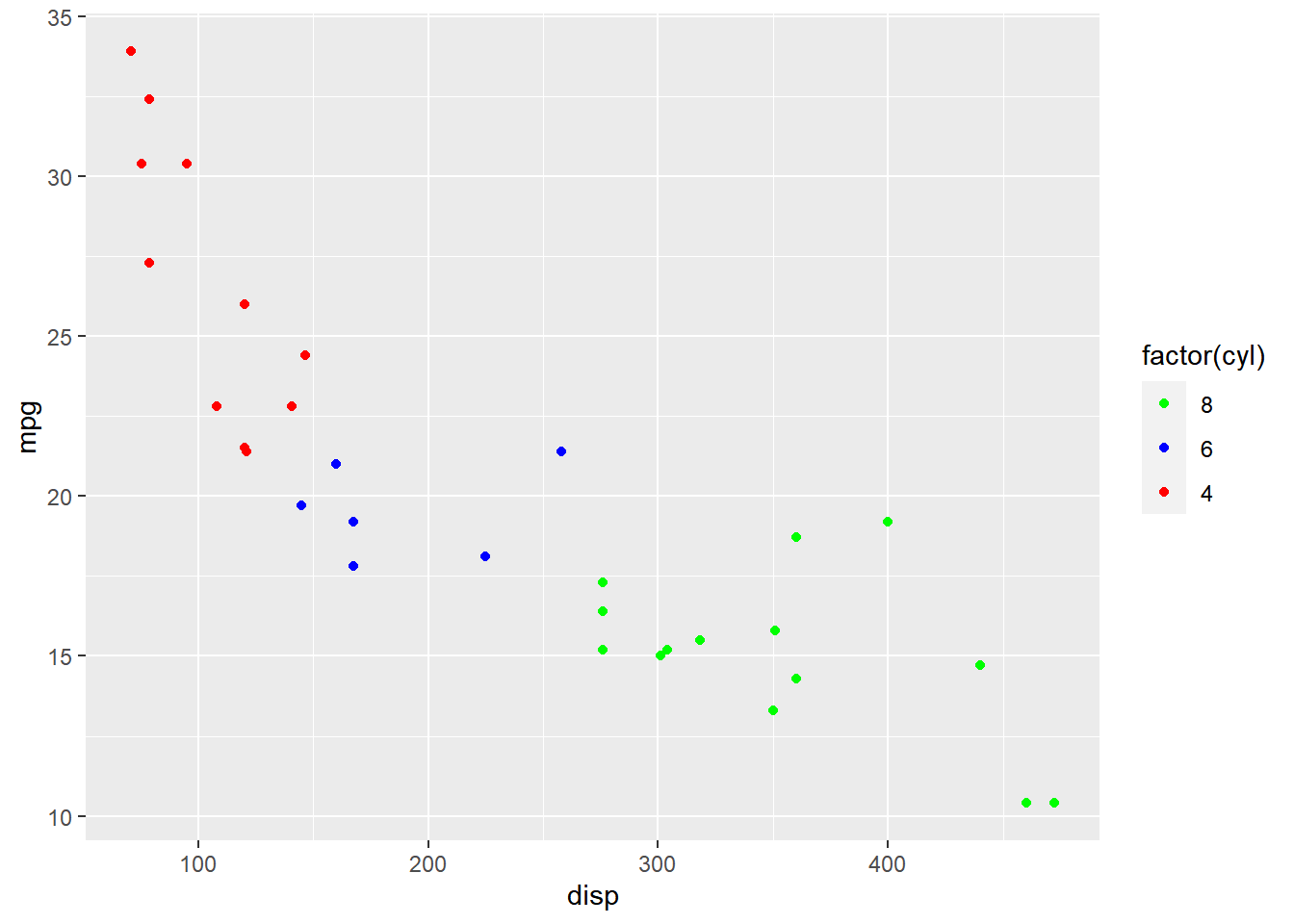#### Putting it all together…

``````ggplot(mtcars) + geom_point(aes(disp, mpg, color = factor(cyl))) +
scale_color_manual(values = c("red", "blue", "green"),
guide = guide_legend(title = "Cylinders", title.hjust = 0.5,
title.position = "top", label.position = "right",
direction = "horizontal", label.hjust = 0.5, nrow = 2, reverse = TRUE)
)``````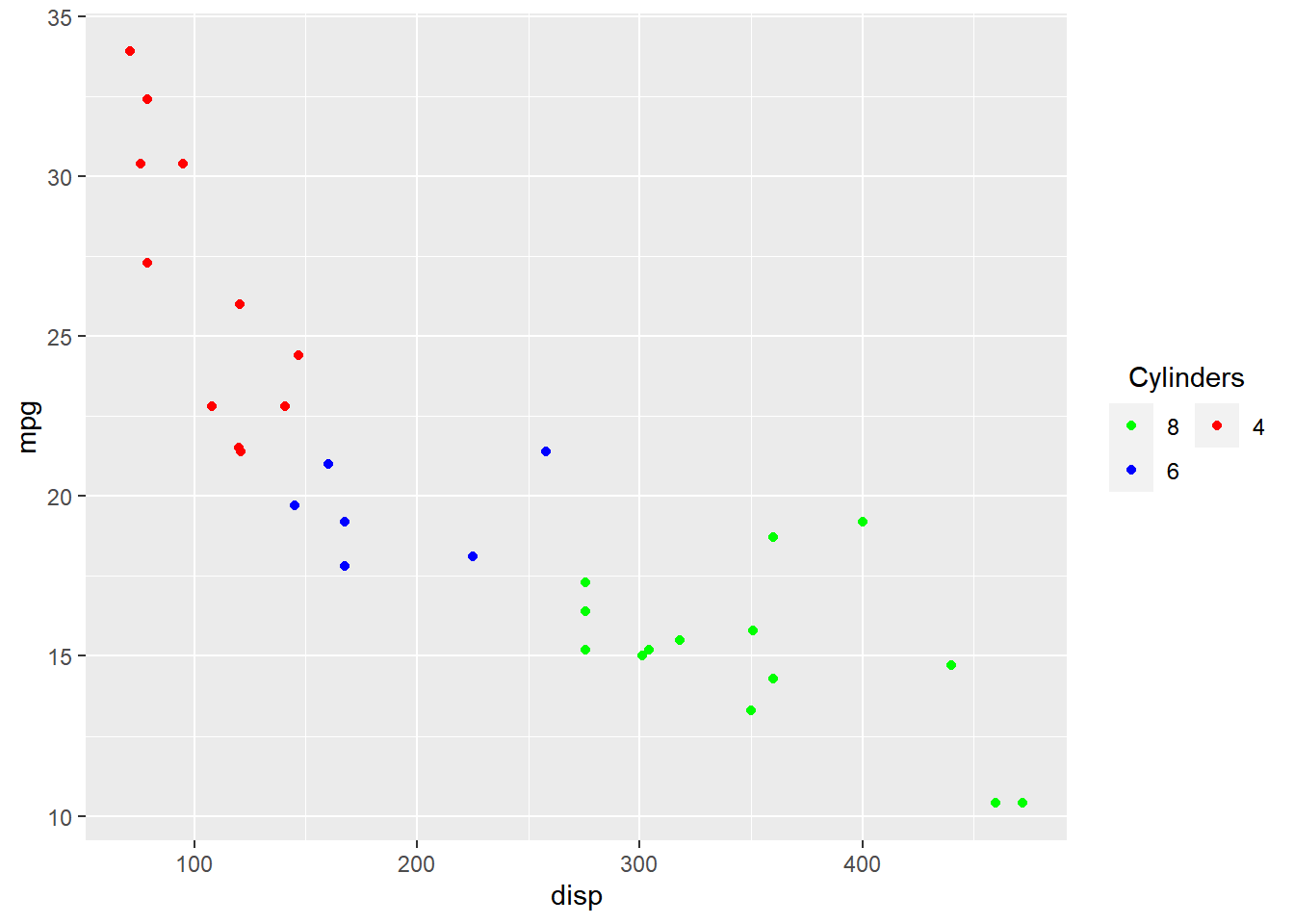### Legend Bar

So far we have looked at modifying components of the legend when it acts as a guide for `color`, `fill` or `shape` i.e. when the aesthetics have been mapped to a categorical variable. In this section, you will learn about `guide_colorbar()` which will allow us to modify the legend when the aesthetics are mapped to a continuous variable.

#### Plot

Let us start with a scatter plot examining the relationship between displacement and miles per gallon from the mtcars data set. We will map the color of the points to the `hp` variable.

``````ggplot(mtcars) +
geom_point(aes(disp, mpg, color = hp))``````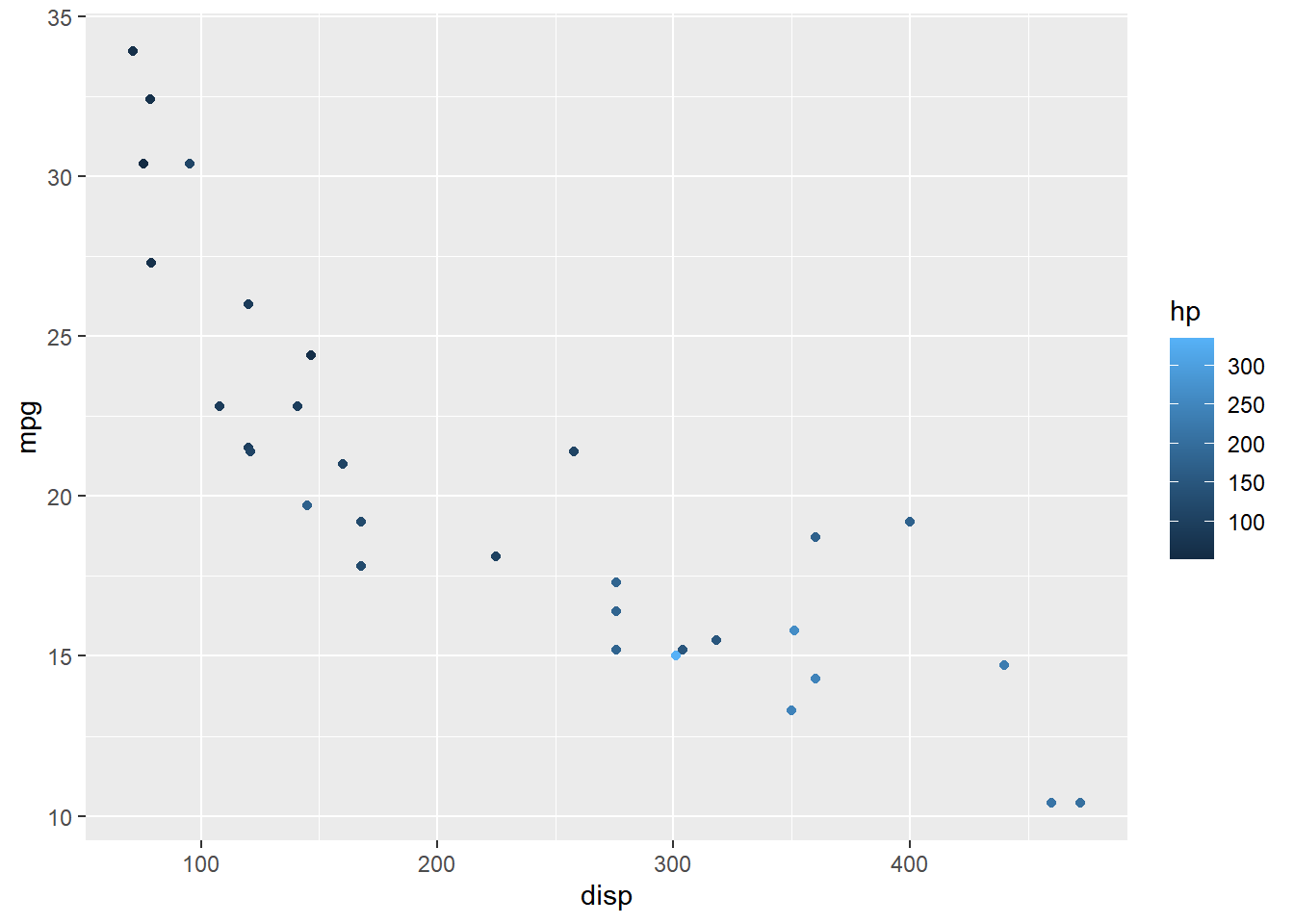#### Width

The width of the bar can be modified using the `barwidth` argument. It is used inside the `guide_colorbar()` function which itself is supplied to the `guide` argument of `scale_color_continuous()`.

``````ggplot(mtcars) +
geom_point(aes(disp, mpg, color = hp)) +
scale_color_continuous(guide = guide_colorbar(
barwidth = 10))``````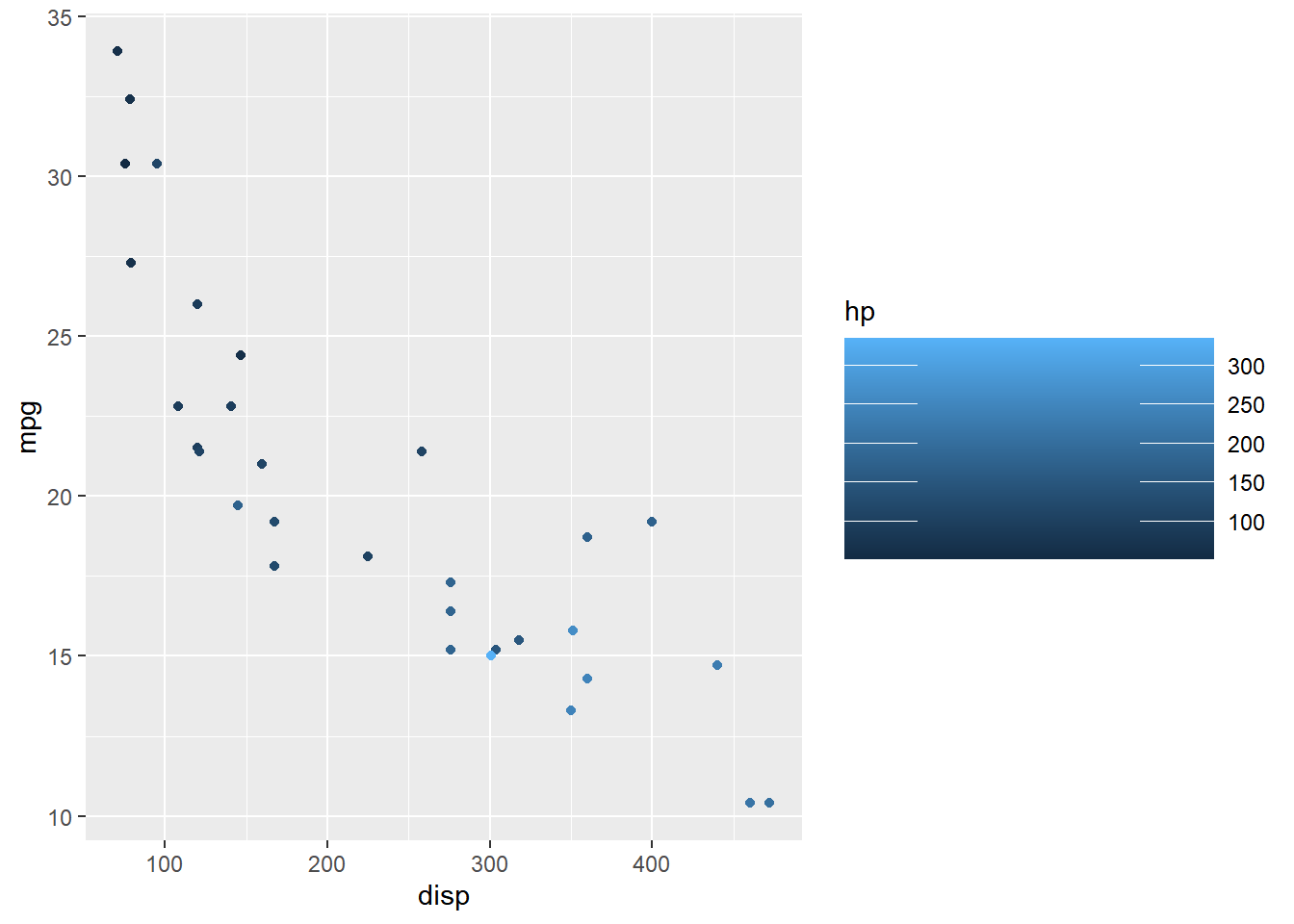#### Height

Similarly, the height of the bar can be modified using the `barheight` argument.

``````ggplot(mtcars) +
geom_point(aes(disp, mpg, color = hp)) +
scale_color_continuous(guide = guide_colorbar(
barheight = 3))``````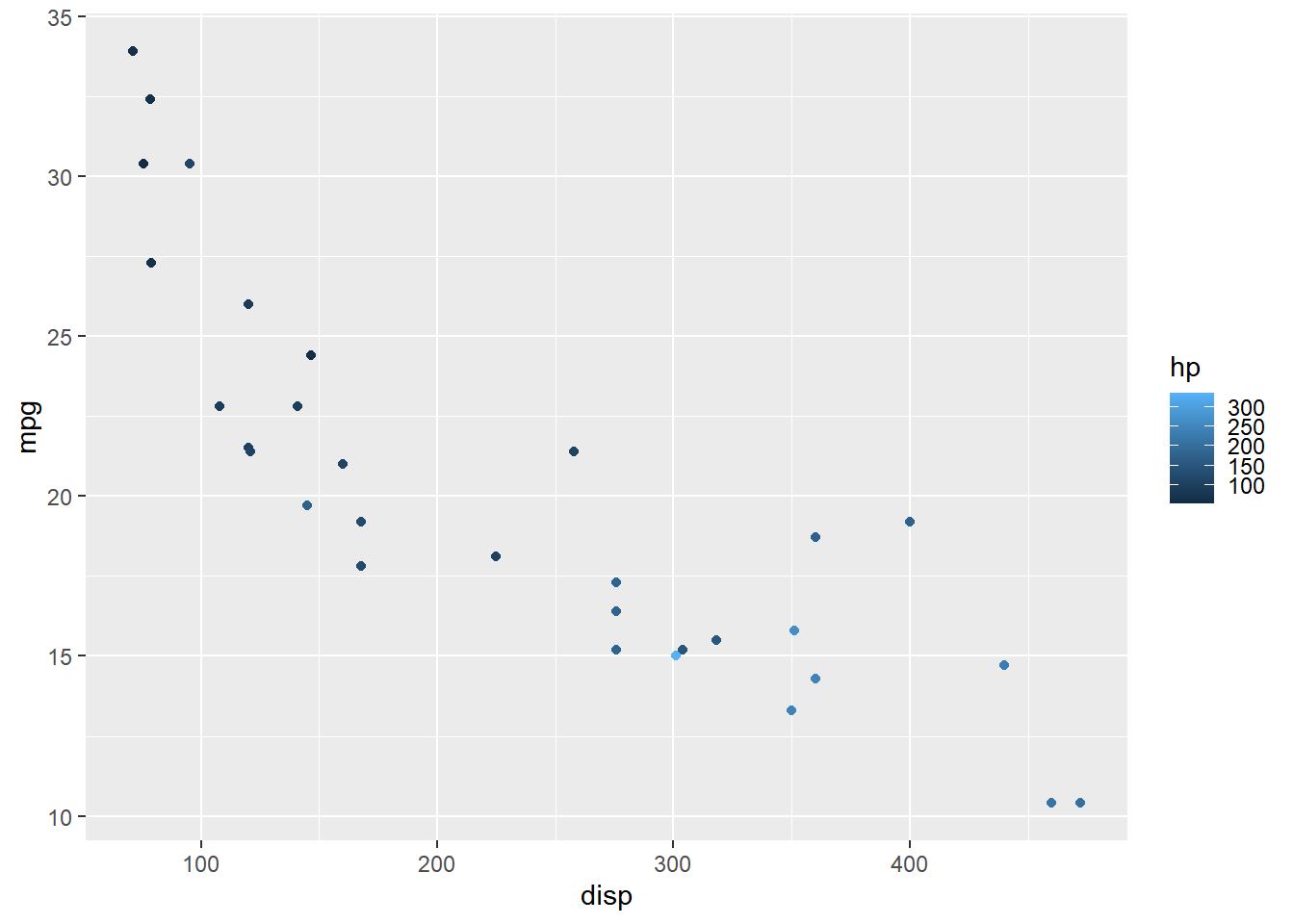#### Bins

The `nbin` argument allows us to specify the number of bins in the bar.

``````ggplot(mtcars) +
geom_point(aes(disp, mpg, color = hp)) +
scale_color_continuous(guide = guide_colorbar(
nbin = 4))``````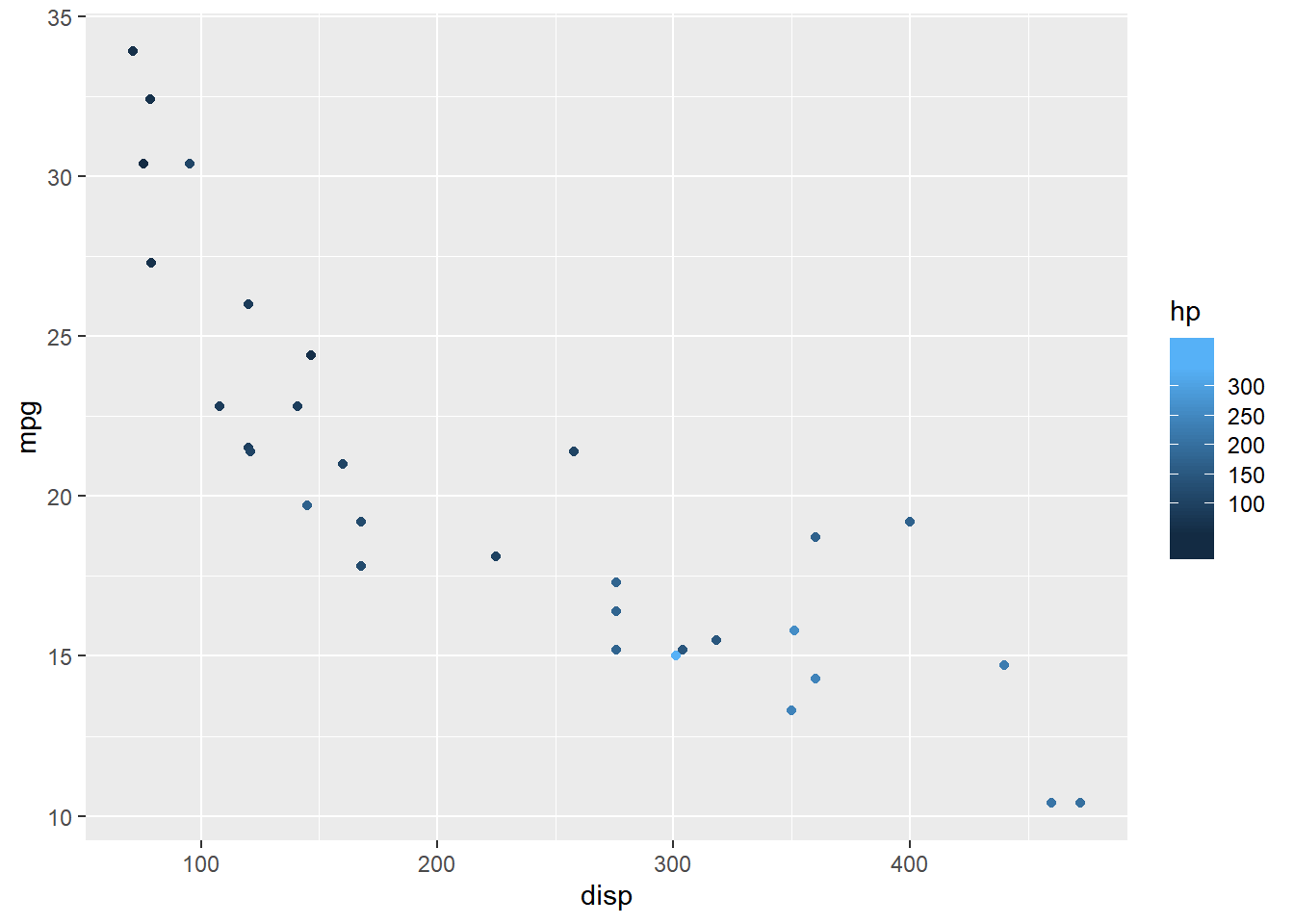#### Ticks

The ticks of the bar can be removed using the `ticks` argument and setting it to `FALSE`.

``````ggplot(mtcars) +
geom_point(aes(disp, mpg, color = hp)) +
scale_color_continuous(guide = guide_colorbar(
ticks = FALSE))``````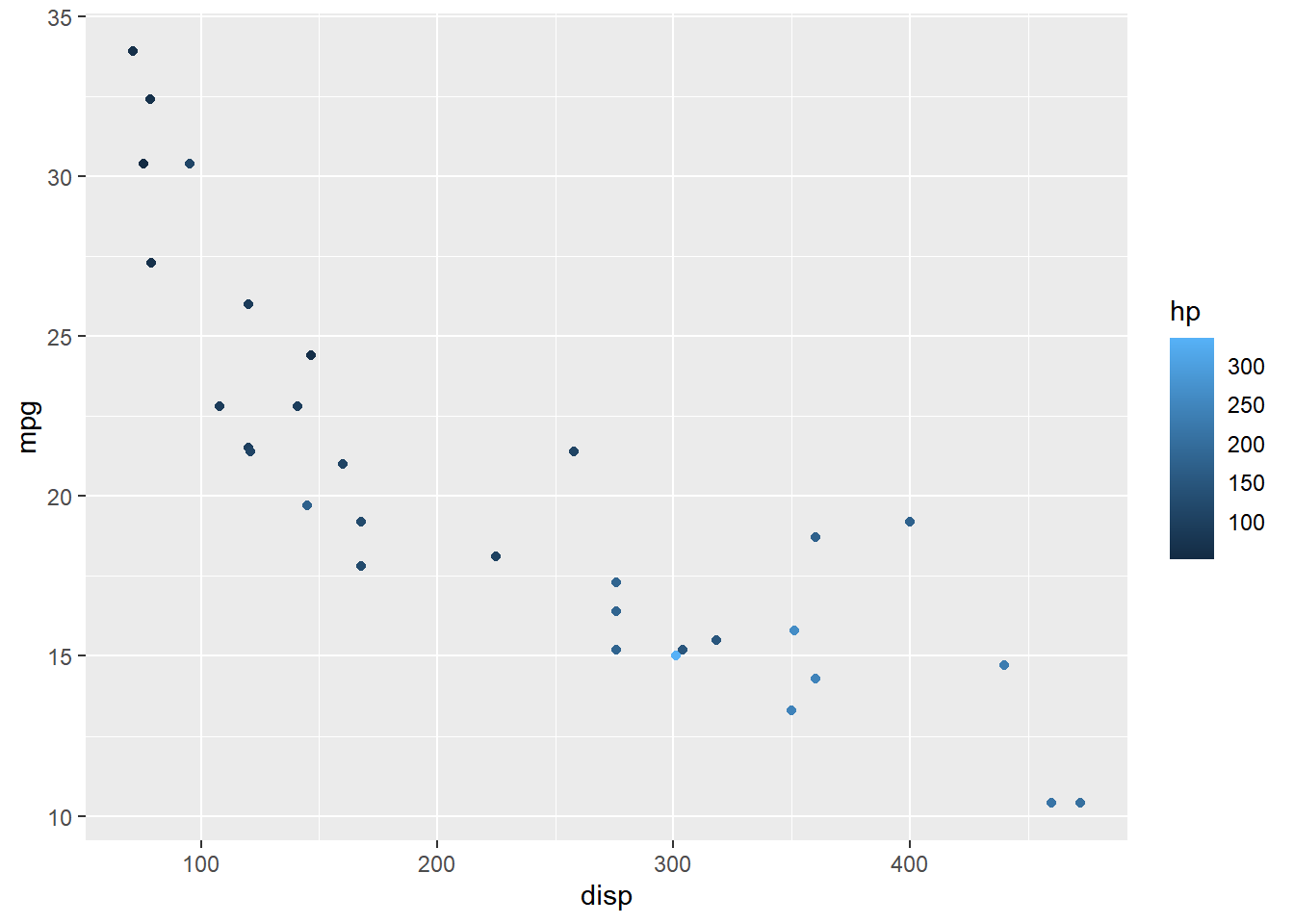#### Upper/Lower Limits

The upper and lower limits of the bars can be drawn or undrawn using the `draw.ulim` and `draw.llim` arguments. They both accept logical values.

``````ggplot(mtcars) +
geom_point(aes(disp, mpg, color = hp)) +
scale_color_continuous(guide = guide_colorbar(
draw.ulim = TRUE, draw.llim = FALSE))``````#### 12.6.0.1 Guides: Color, Shape & Size

The `guides()` function can be used to create multiple legends to act as a guide for `color`, `shape`, `size` etc. as shown below. First, we map color, shape and size to different variables. Next, in the `guides()` function, we supply values to each of the above aesthetics to indicate the type of legend.

``````ggplot(mtcars) +
geom_point(aes(disp, mpg, color = hp,
size = qsec, shape = factor(gear))) +
guides(color = "colorbar", shape = "legend", size = "legend")``````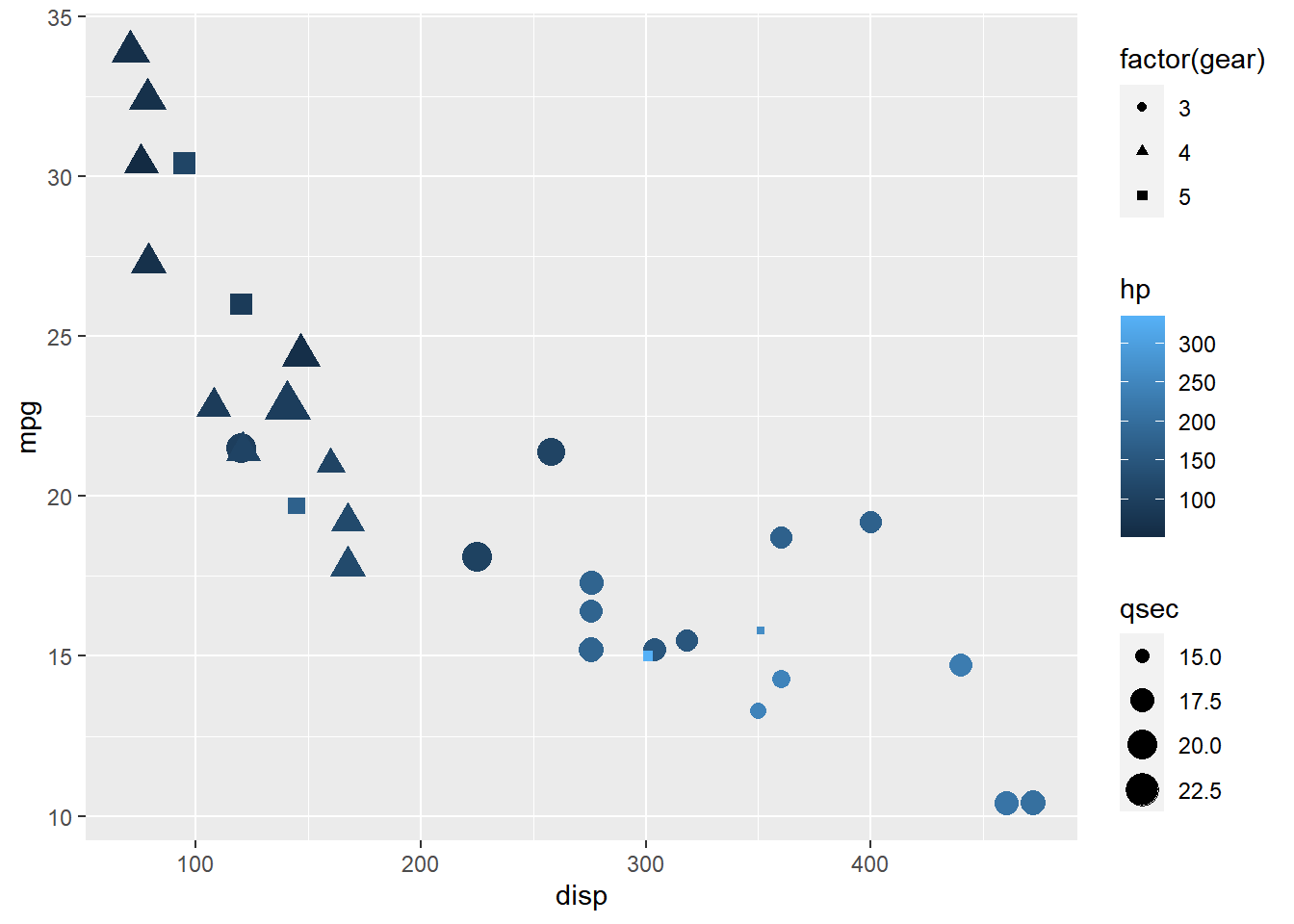#### Guides: Title

To modify the components of the different legends, we must use the `guide_*` family of functions. In the below example, we use `guide_colorbar()` for the legend acting as guide for color mapped to a continuous variable and `guide_legend()` for the legends acting as guide for shape/size mapped to categorical variables.

``````ggplot(mtcars) +
geom_point(aes(disp, mpg, color = hp, size = wt, shape = factor(gear))) +
guides(color = guide_colorbar(title = "Horsepower"),
shape = guide_legend(title = "Weight"), size = guide_legend(title = "Gear")
)``````